Скачать презентацию Set 8 Momentum 1 Today There is

e211754f7146928f24973f8182de06d8.ppt

• Количество слайдов: 84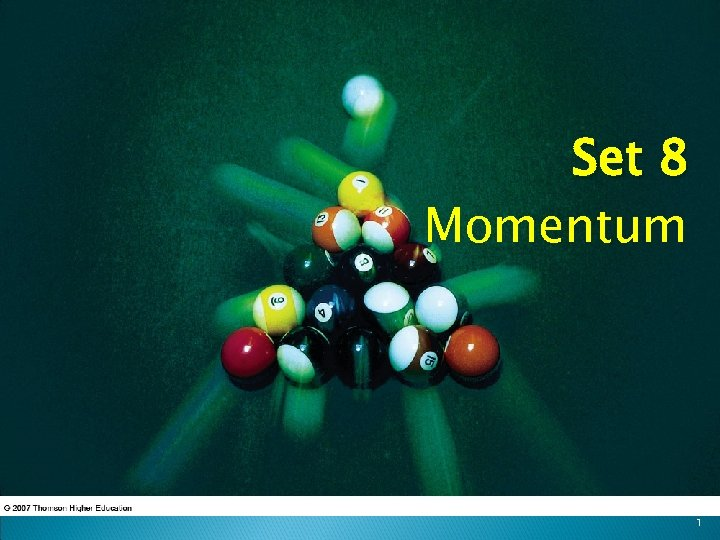Set 8 Momentum 1Today There is a new (and easy) Web. Assign posted. We begin a new chapter (6) in the textbook. ◦ MOMENTUM There is a big connection to music that will become clear. We will come back to one of the skipped chapters later. There will be a Web. Assignment on this material as well, so don’t delay with the current assignment. More on your grades on Monday. 2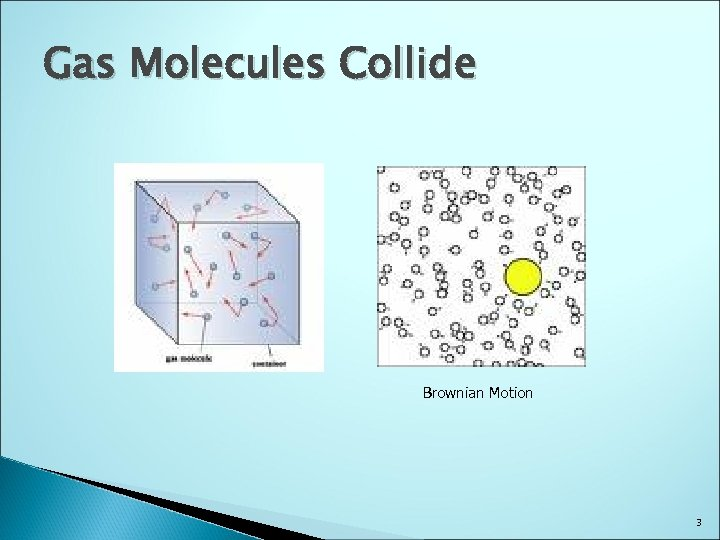Gas Molecules Collide Brownian Motion 3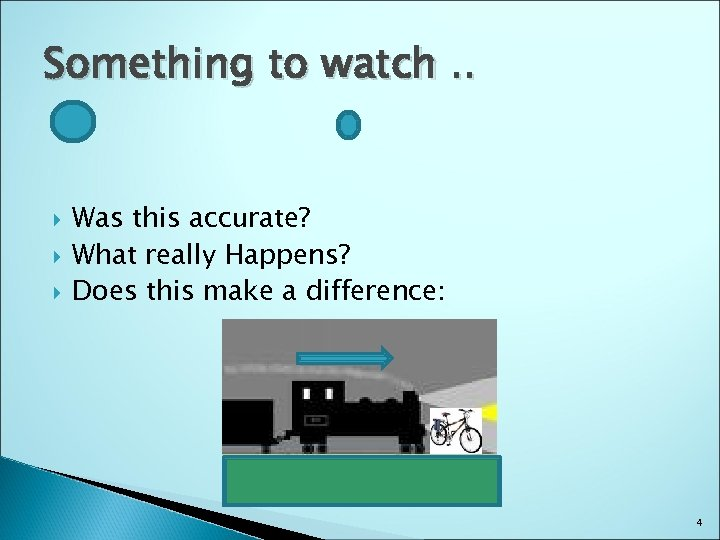Something to watch. . Was this accurate? What really Happens? Does this make a difference: 4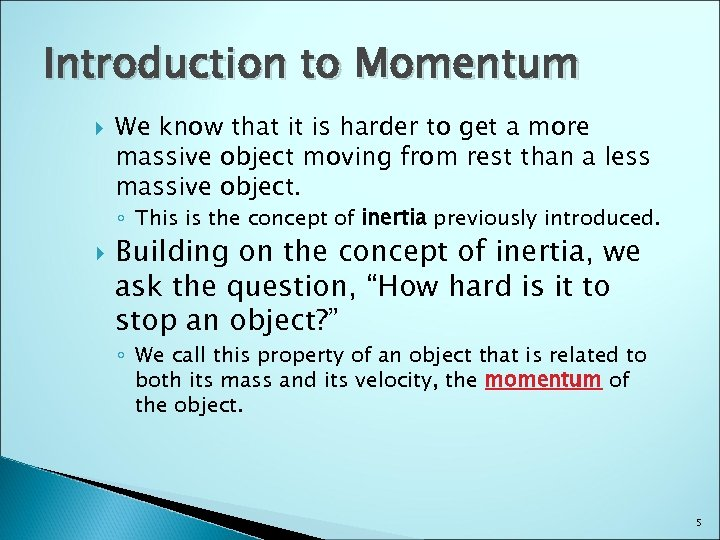Introduction to Momentum We know that it is harder to get a more massive object moving from rest than a less massive object. ◦ This is the concept of inertia previously introduced. Building on the concept of inertia, we ask the question, “How hard is it to stop an object? ” ◦ We call this property of an object that is related to both its mass and its velocity, the momentum of the object. 5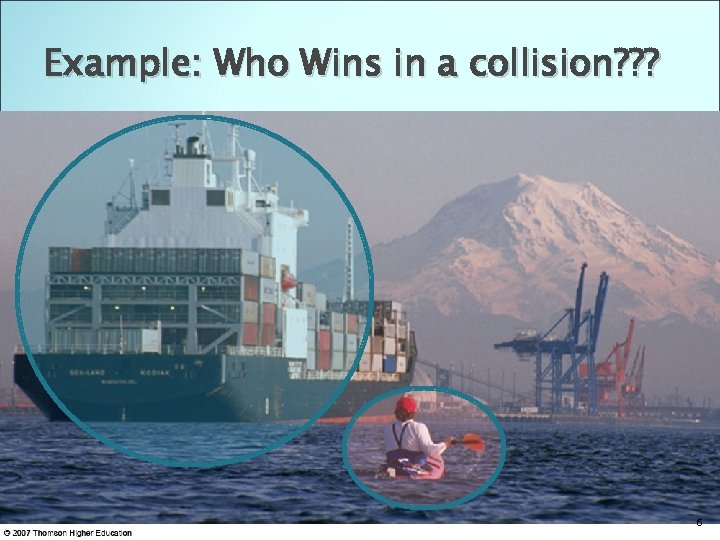Example: Who Wins in a collision? ? ? 6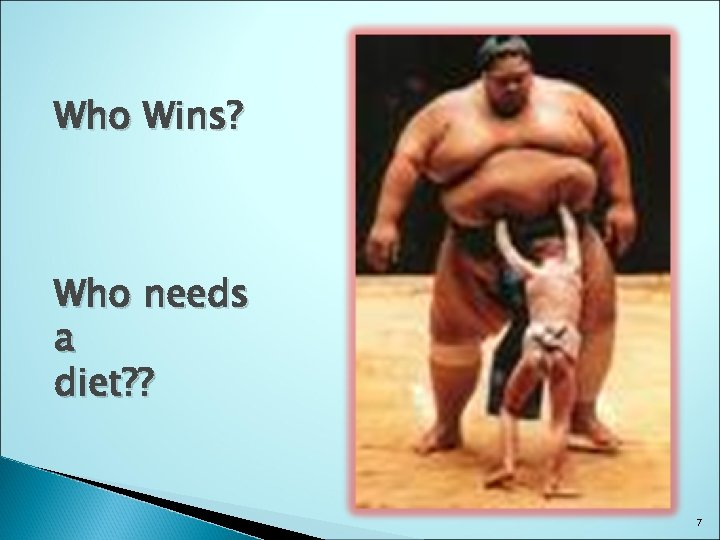Who Wins? Who needs a diet? ? 7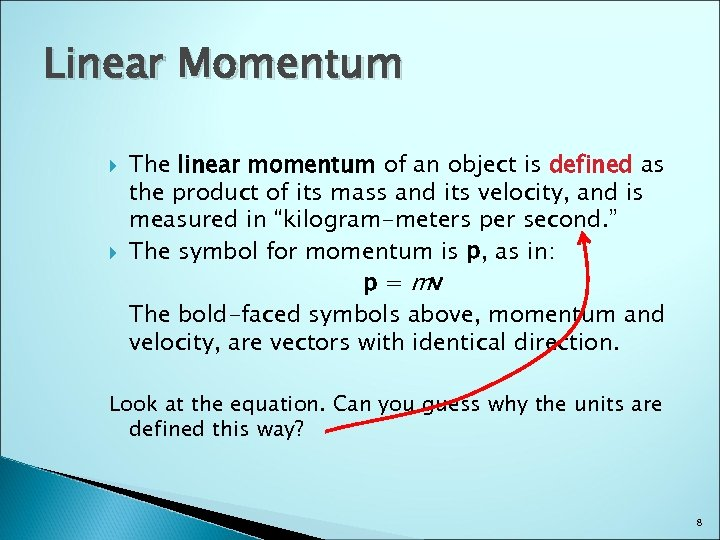Linear Momentum The linear momentum of an object is defined as the product of its mass and its velocity, and is measured in “kilogram-meters per second. ” The symbol for momentum is p, as in: p = mv The bold-faced symbols above, momentum and velocity, are vectors with identical direction. Look at the equation. Can you guess why the units are defined this way? 8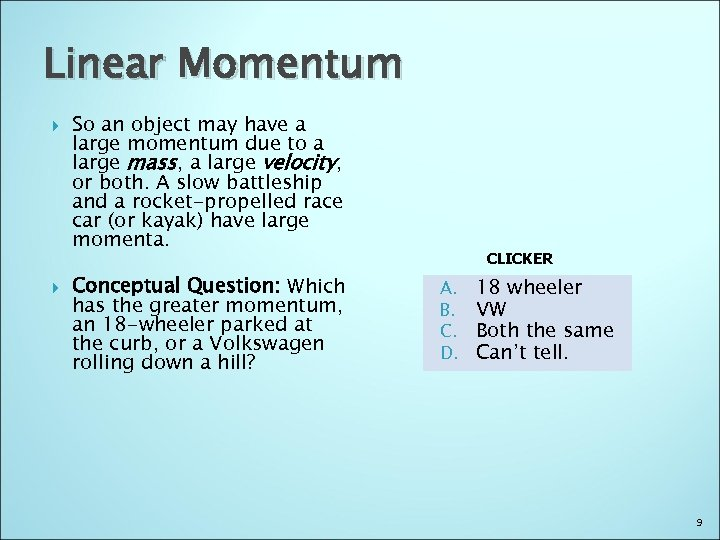Linear Momentum So an object may have a large momentum due to a large mass, a large velocity, or both. A slow battleship and a rocket-propelled race car (or kayak) have large momenta. Conceptual Question: Which has the greater momentum, an 18 -wheeler parked at the curb, or a Volkswagen rolling down a hill? CLICKER A. B. C. D. 18 wheeler VW Both the same Can’t tell. 9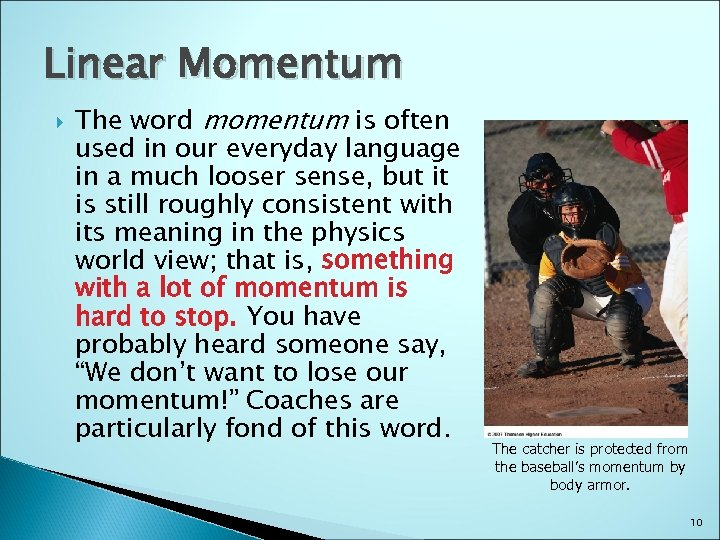Linear Momentum The word momentum is often used in our everyday language in a much looser sense, but it is still roughly consistent with its meaning in the physics world view; that is, something with a lot of momentum is hard to stop. You have probably heard someone say, “We don’t want to lose our momentum!” Coaches are particularly fond of this word. The catcher is protected from the baseball’s momentum by body armor. 10What’s being transferred? 11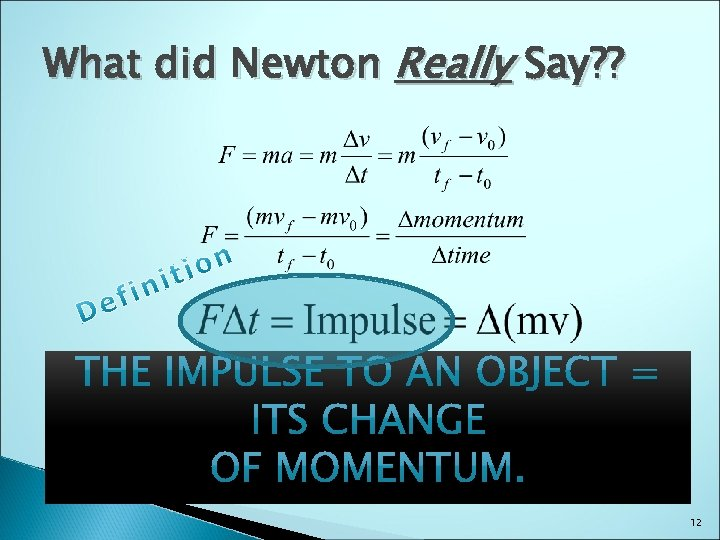What did Newton Really Say? ? 12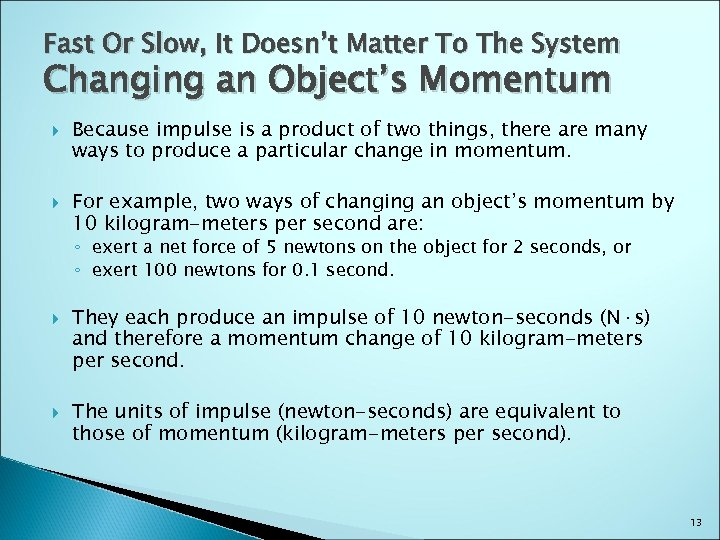Fast Or Slow, It Doesn’t Matter To The System Changing an Object’s Momentum Because impulse is a product of two things, there are many ways to produce a particular change in momentum. For example, two ways of changing an object’s momentum by 10 kilogram-meters per second are: ◦ exert a net force of 5 newtons on the object for 2 seconds, or ◦ exert 100 newtons for 0. 1 second. They each produce an impulse of 10 newton-seconds (N·s) and therefore a momentum change of 10 kilogram-meters per second. The units of impulse (newton-seconds) are equivalent to those of momentum (kilogram-meters per second). 13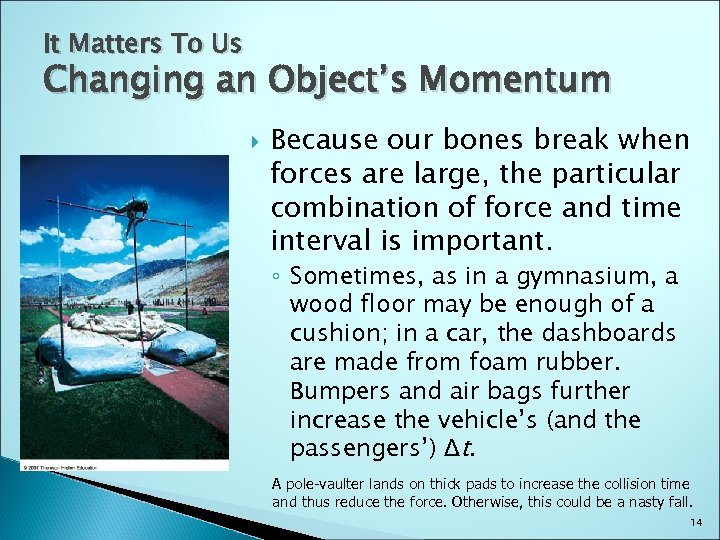It Matters To Us Changing an Object’s Momentum Because our bones break when forces are large, the particular combination of force and time interval is important. ◦ Sometimes, as in a gymnasium, a wood floor may be enough of a cushion; in a car, the dashboards are made from foam rubber. Bumpers and air bags further increase the vehicle’s (and the passengers’) Δt. A pole-vaulter lands on thick pads to increase the collision time and thus reduce the force. Otherwise, this could be a nasty fall. 14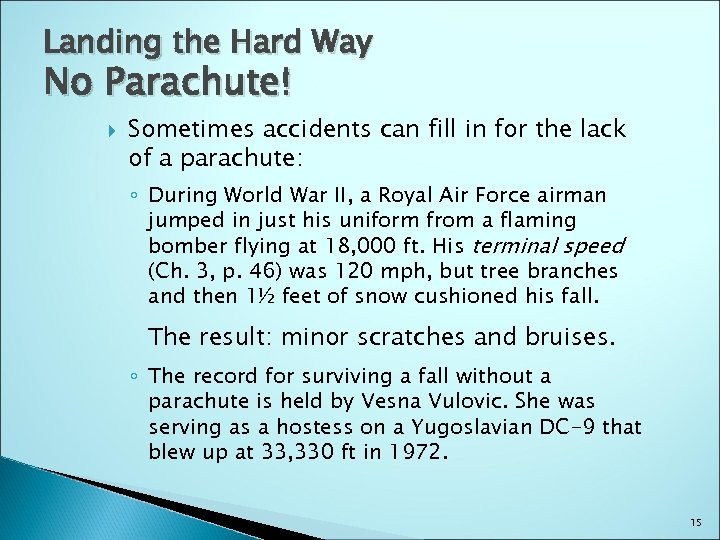Landing the Hard Way No Parachute! Sometimes accidents can fill in for the lack of a parachute: ◦ During World War II, a Royal Air Force airman jumped in just his uniform from a flaming bomber flying at 18, 000 ft. His terminal speed (Ch. 3, p. 46) was 120 mph, but tree branches and then 1½ feet of snow cushioned his fall. The result: minor scratches and bruises. ◦ The record for surviving a fall without a parachute is held by Vesna Vulovic. She was serving as a hostess on a Yugoslavian DC-9 that blew up at 33, 330 ft in 1972. 15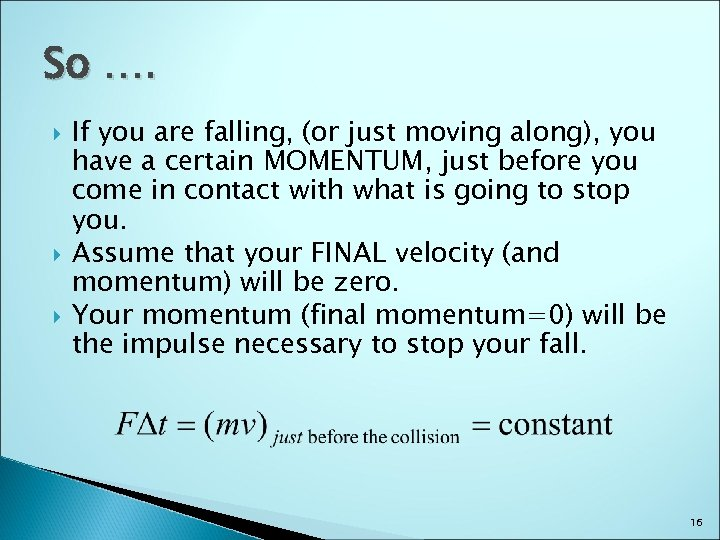So …. If you are falling, (or just moving along), you have a certain MOMENTUM, just before you come in contact with what is going to stop you. Assume that your FINAL velocity (and momentum) will be zero. Your momentum (final momentum=0) will be the impulse necessary to stop your fall. 16AND Smaller Dt Bigger Force 1718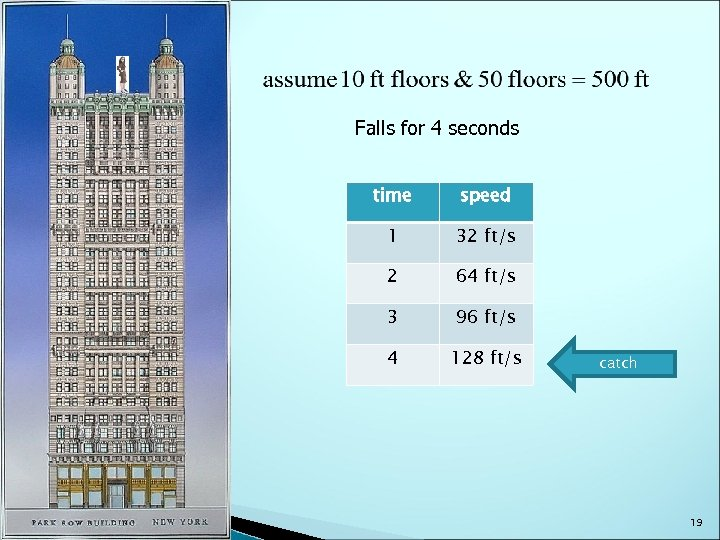Falls for 4 seconds time speed 1 32 ft/s 2 64 ft/s 3 96 ft/s 4 128 ft/s catch 19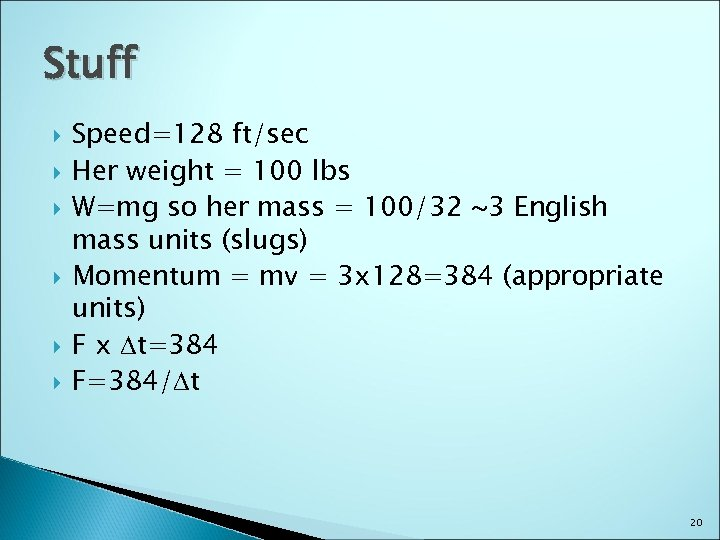Stuff Speed=128 ft/sec Her weight = 100 lbs W=mg so her mass = 100/32 ~3 English mass units (slugs) Momentum = mv = 3 x 128=384 (appropriate units) F x Dt=384 F=384/Dt 20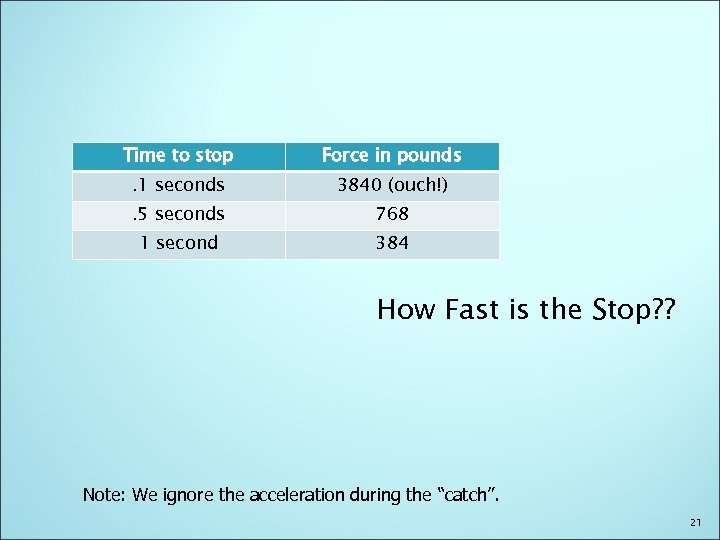Time to stop Force in pounds . 1 seconds 3840 (ouch!) . 5 seconds 768 1 second 384 How Fast is the Stop? ? Note: We ignore the acceleration during the “catch”. 2122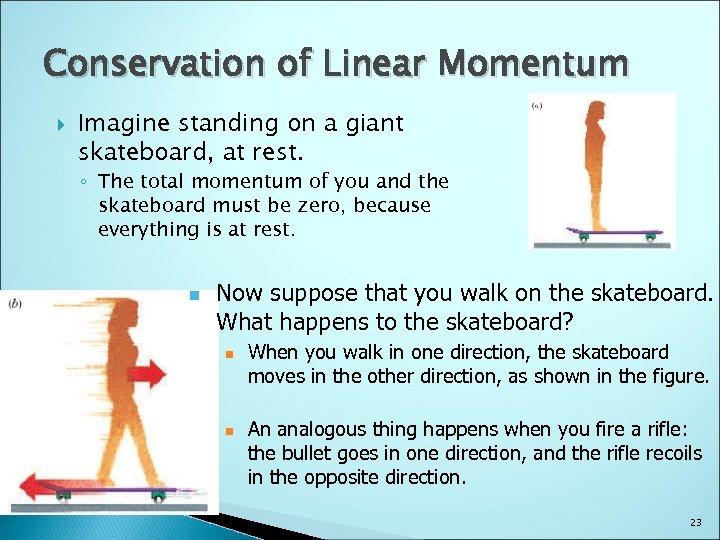Conservation of Linear Momentum Imagine standing on a giant skateboard, at rest. ◦ The total momentum of you and the skateboard must be zero, because everything is at rest. n Now suppose that you walk on the skateboard. What happens to the skateboard? n n When you walk in one direction, the skateboard moves in the other direction, as shown in the figure. An analogous thing happens when you fire a rifle: the bullet goes in one direction, and the rifle recoils in the opposite direction. 23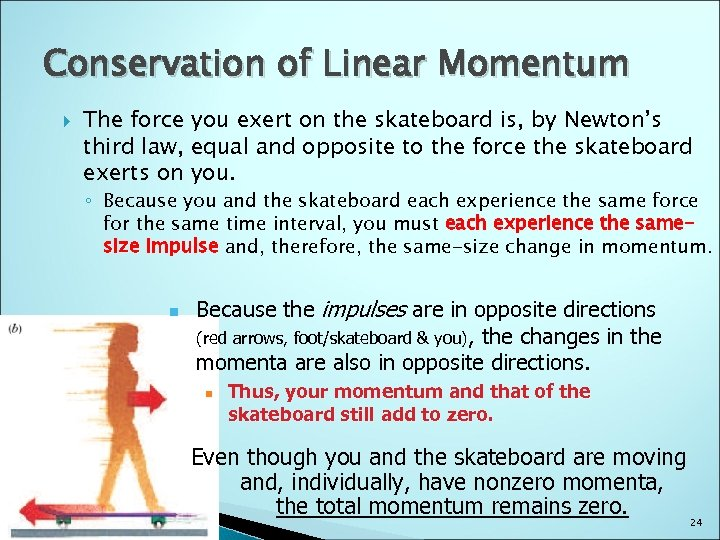Conservation of Linear Momentum The force you exert on the skateboard is, by Newton’s third law, equal and opposite to the force the skateboard exerts on you. ◦ Because you and the skateboard each experience the same force for the same time interval, you must each experience the samesize impulse and, therefore, the same-size change in momentum. n Because the impulses are in opposite directions (red arrows, foot/skateboard & you), the changes in the momenta are also in opposite directions. n Thus, your momentum and that of the skateboard still add to zero. Even though you and the skateboard are moving and, individually, have nonzero momenta, the total momentum remains zero. 24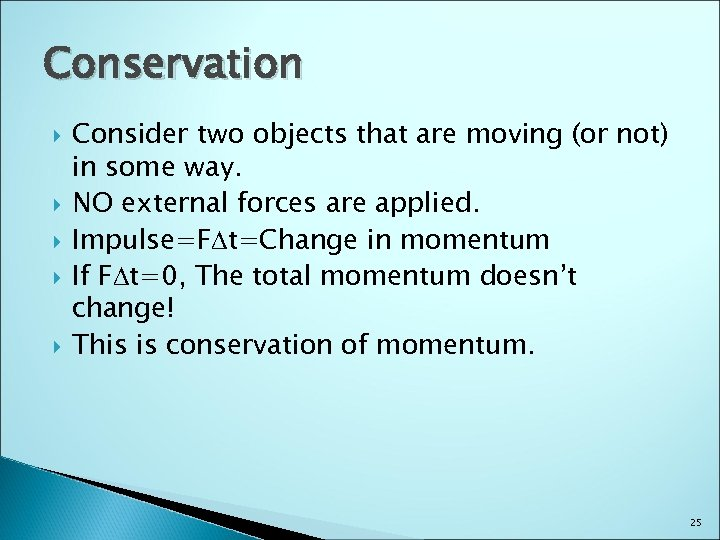Conservation Consider two objects that are moving (or not) in some way. NO external forces are applied. Impulse=FDt=Change in momentum If FDt=0, The total momentum doesn’t change! This is conservation of momentum. 25Need a Volunteer Scateboarder HEAVY!! 26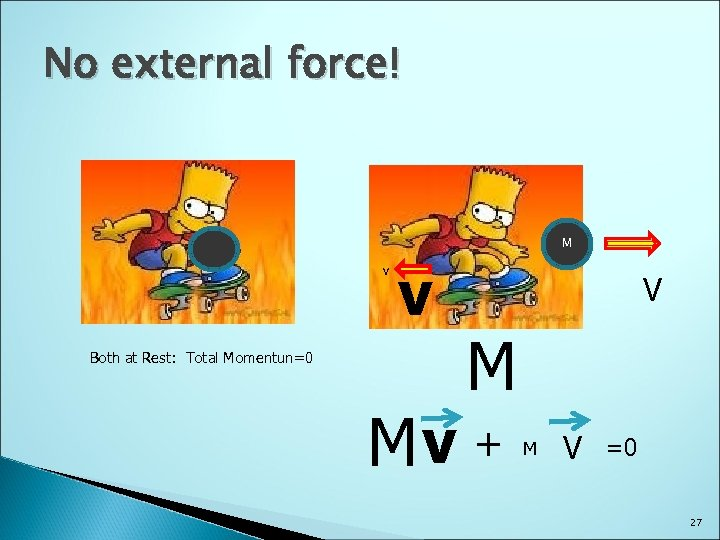No external force! M v Both at Rest: Total Momentun=0 v V M Mv + M V =0 27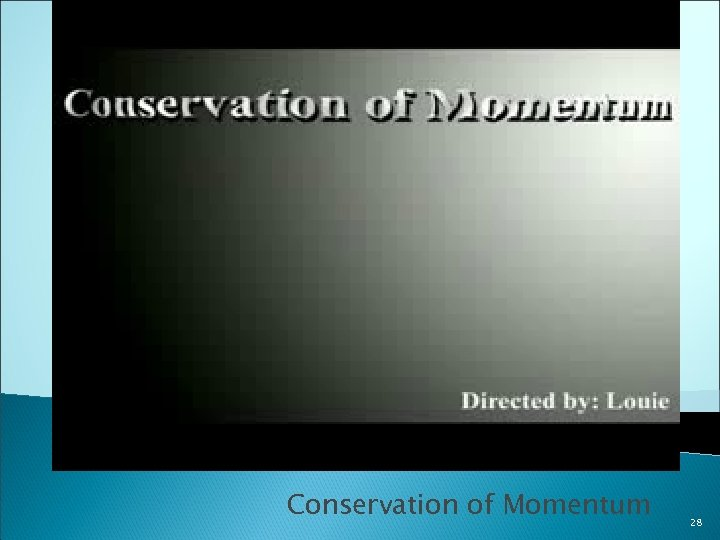http: //www. youtube. com/watch? v=KL 8 -Pbd. RYY 0 Conservation of Momentum 28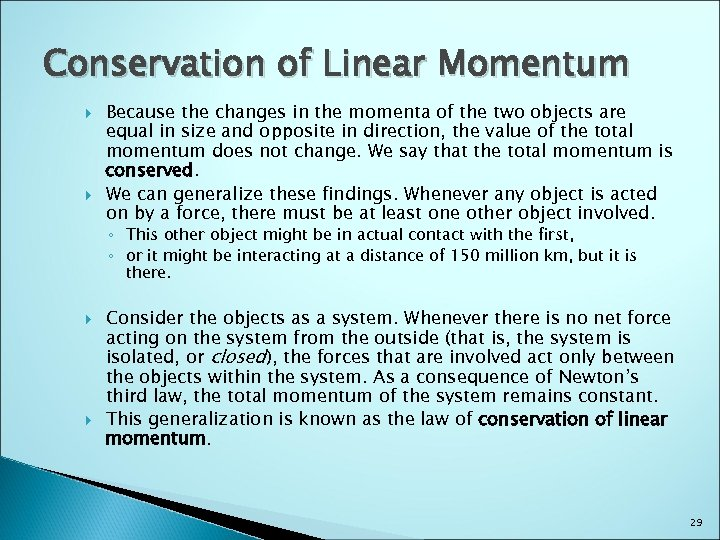Conservation of Linear Momentum Because the changes in the momenta of the two objects are equal in size and opposite in direction, the value of the total momentum does not change. We say that the total momentum is conserved. We can generalize these findings. Whenever any object is acted on by a force, there must be at least one other object involved. ◦ This other object might be in actual contact with the first, ◦ or it might be interacting at a distance of 150 million km, but it is there. Consider the objects as a system. Whenever there is no net force acting on the system from the outside (that is, the system is isolated, or closed), the forces that are involved act only between the objects within the system. As a consequence of Newton’s third law, the total momentum of the system remains constant. This generalization is known as the law of conservation of linear momentum. 29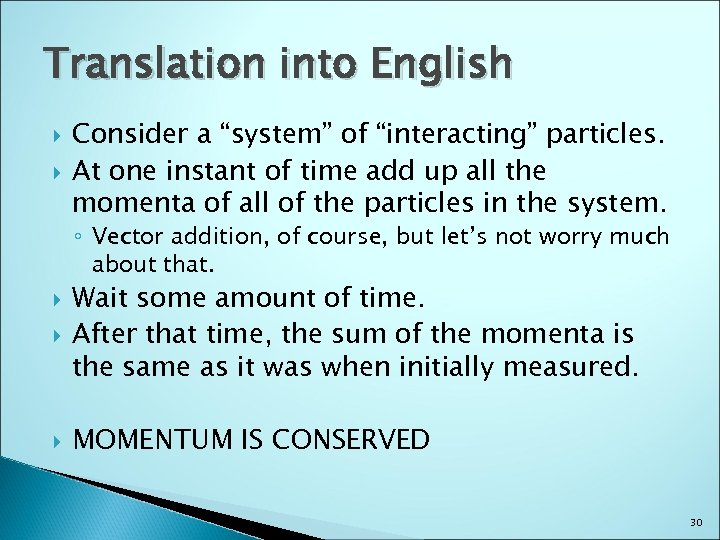Translation into English Consider a “system” of “interacting” particles. At one instant of time add up all the momenta of all of the particles in the system. ◦ Vector addition, of course, but let’s not worry much about that. Wait some amount of time. After that time, the sum of the momenta is the same as it was when initially measured. MOMENTUM IS CONSERVED 30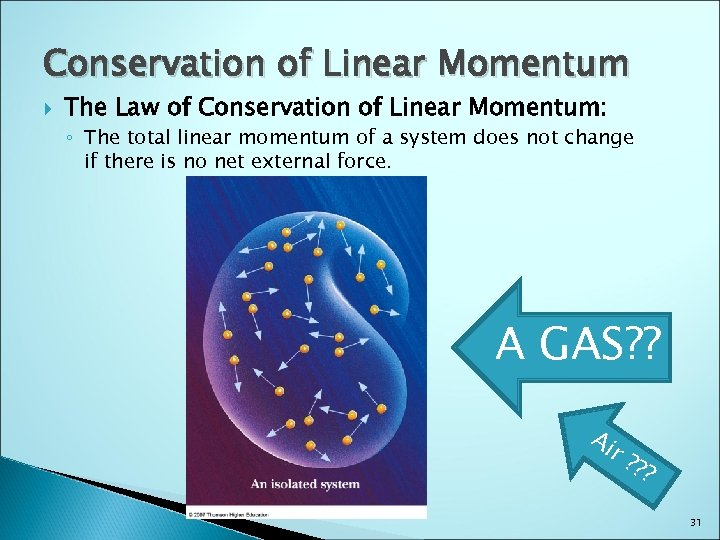Conservation of Linear Momentum The Law of Conservation of Linear Momentum: ◦ The total linear momentum of a system does not change if there is no net external force. A GAS? ? Ai r? ? ? 31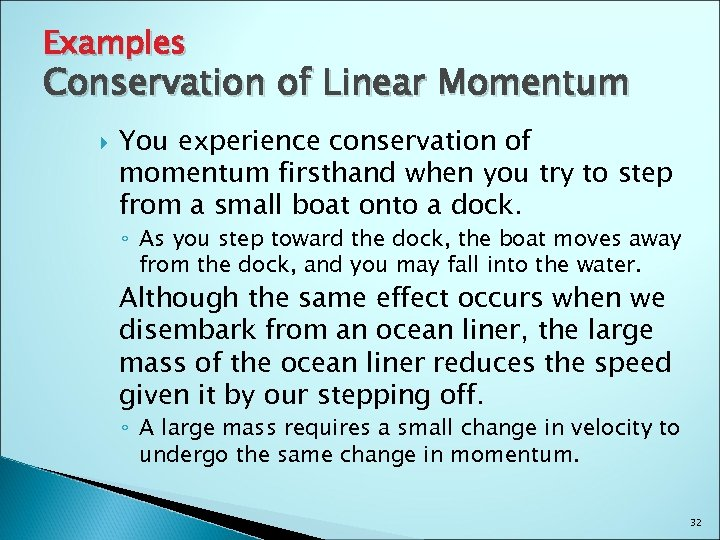Examples Conservation of Linear Momentum You experience conservation of momentum firsthand when you try to step from a small boat onto a dock. ◦ As you step toward the dock, the boat moves away from the dock, and you may fall into the water. Although the same effect occurs when we disembark from an ocean liner, the large mass of the ocean liner reduces the speed given it by our stepping off. ◦ A large mass requires a small change in velocity to undergo the same change in momentum. 32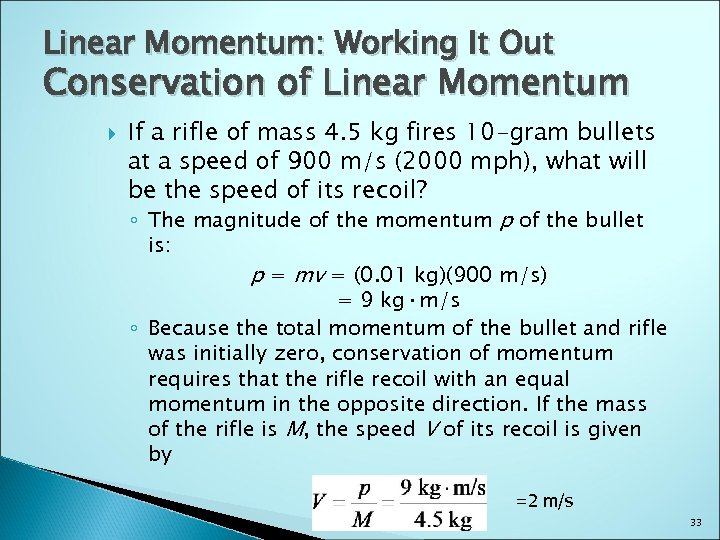Linear Momentum: Working It Out Conservation of Linear Momentum If a rifle of mass 4. 5 kg fires 10 -gram bullets at a speed of 900 m/s (2000 mph), what will be the speed of its recoil? ◦ The magnitude of the momentum p of the bullet is: p = mv = (0. 01 kg)(900 m/s) = 9 kg·m/s ◦ Because the total momentum of the bullet and rifle was initially zero, conservation of momentum requires that the rifle recoil with an equal momentum in the opposite direction. If the mass of the rifle is M, the speed V of its recoil is given by =2 m/s 33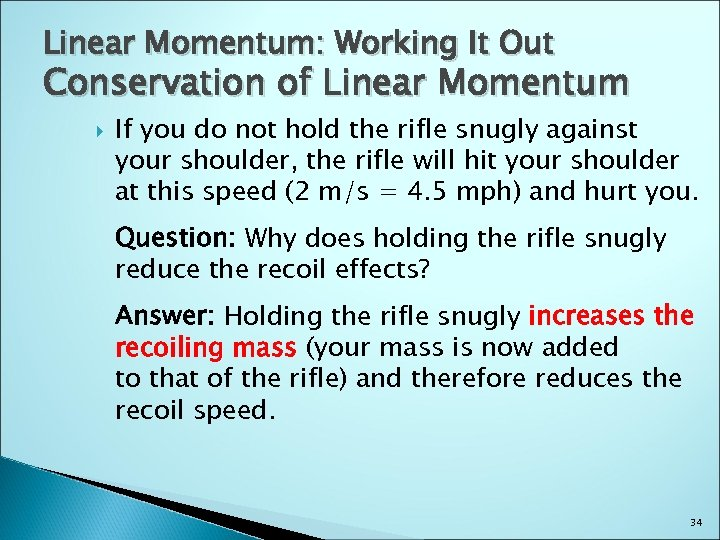Linear Momentum: Working It Out Conservation of Linear Momentum If you do not hold the rifle snugly against your shoulder, the rifle will hit your shoulder at this speed (2 m/s = 4. 5 mph) and hurt you. Question: Why does holding the rifle snugly reduce the recoil effects? Answer: Holding the rifle snugly increases the recoiling mass (your mass is now added to that of the rifle) and therefore reduces the recoil speed. 34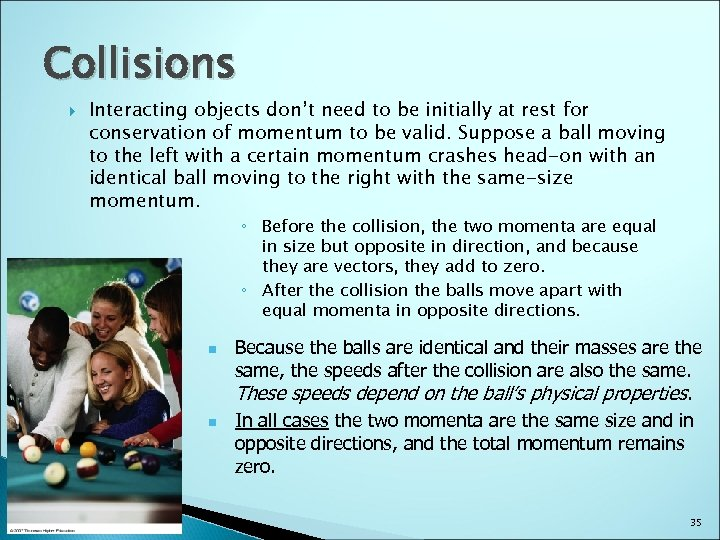Collisions Interacting objects don’t need to be initially at rest for conservation of momentum to be valid. Suppose a ball moving to the left with a certain momentum crashes head-on with an identical ball moving to the right with the same-size momentum. ◦ Before the collision, the two momenta are equal in size but opposite in direction, and because they are vectors, they add to zero. ◦ After the collision the balls move apart with equal momenta in opposite directions. n n Because the balls are identical and their masses are the same, the speeds after the collision are also the same. These speeds depend on the ball’s physical properties. In all cases the two momenta are the same size and in opposite directions, and the total momentum remains zero. 35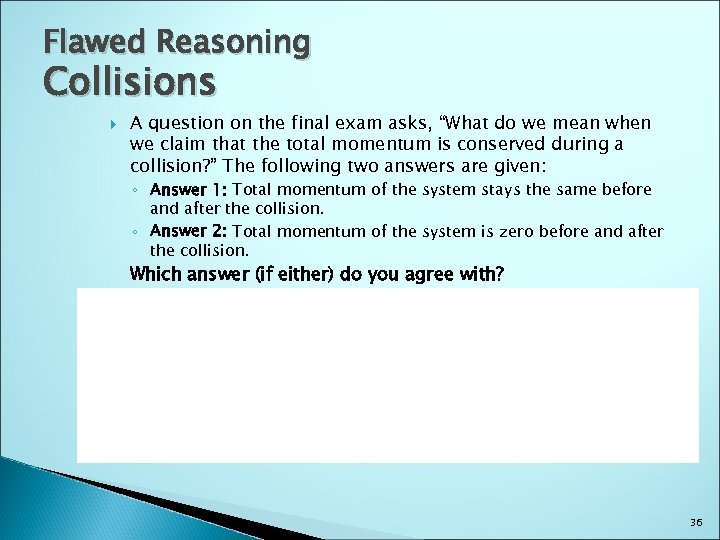Flawed Reasoning Collisions A question on the final exam asks, “What do we mean when we claim that the total momentum is conserved during a collision? ” The following two answers are given: ◦ Answer 1: Total momentum of the system stays the same before and after the collision. ◦ Answer 2: Total momentum of the system is zero before and after the collision. Which answer (if either) do you agree with? Answer: While we have considered several examples in which the total momentum of the system is zero, this is not the most general case. The momentum of a system can have any magnitude and any direction before the collision. If momentum is conserved, the momentum of the system always has the same magnitude and direction after the collision. Therefore, answer 1 is correct. This is a very powerful principle because of the word always. 36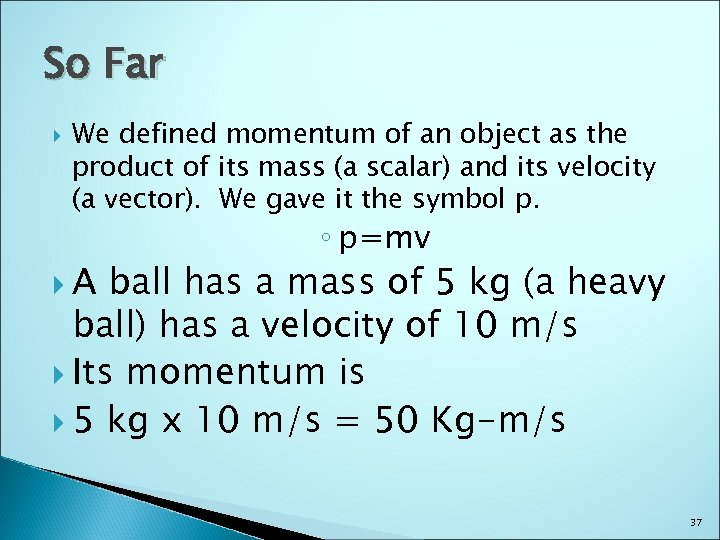So Far We defined momentum of an object as the product of its mass (a scalar) and its velocity (a vector). We gave it the symbol p. A ◦ p=mv ball has a mass of 5 kg (a heavy ball) has a velocity of 10 m/s Its momentum is 5 kg x 10 m/s = 50 Kg-m/s 37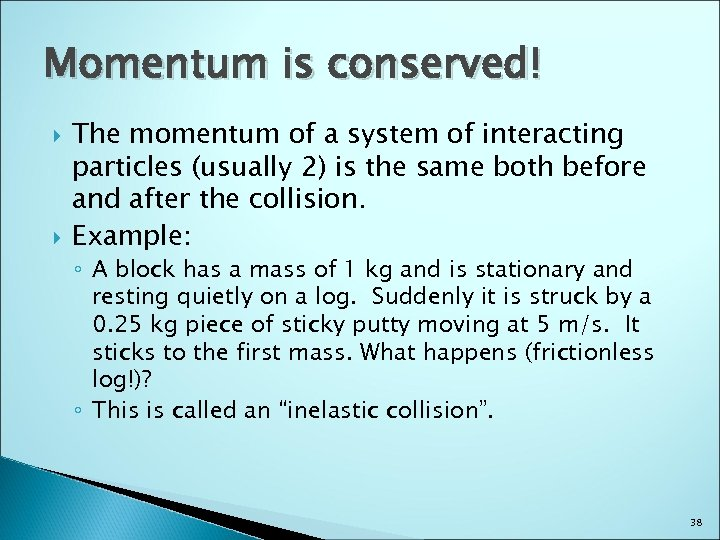Momentum is conserved! The momentum of a system of interacting particles (usually 2) is the same both before and after the collision. Example: ◦ A block has a mass of 1 kg and is stationary and resting quietly on a log. Suddenly it is struck by a 0. 25 kg piece of sticky putty moving at 5 m/s. It sticks to the first mass. What happens (frictionless log!)? ◦ This is called an “inelastic collision”. 38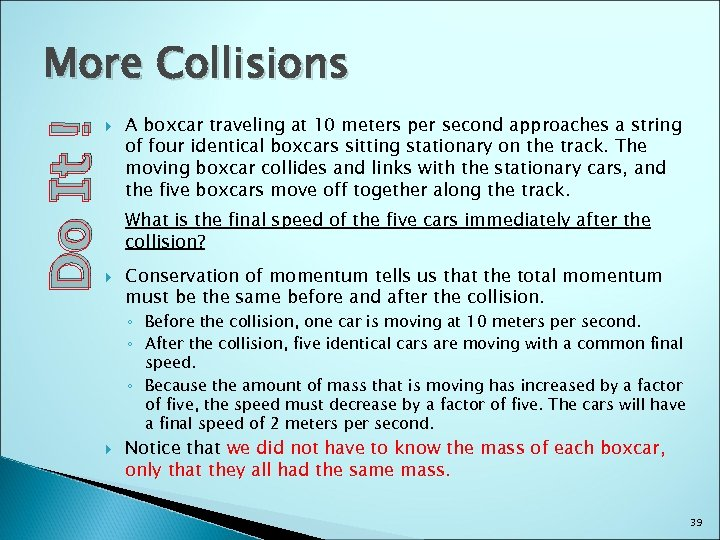Do It ! More Collisions A boxcar traveling at 10 meters per second approaches a string of four identical boxcars sitting stationary on the track. The moving boxcar collides and links with the stationary cars, and the five boxcars move off together along the track. What is the final speed of the five cars immediately after the collision? Conservation of momentum tells us that the total momentum must be the same before and after the collision. ◦ Before the collision, one car is moving at 10 meters per second. ◦ After the collision, five identical cars are moving with a common final speed. ◦ Because the amount of mass that is moving has increased by a factor of five, the speed must decrease by a factor of five. The cars will have a final speed of 2 meters per second. Notice that we did not have to know the mass of each boxcar, only that they all had the same mass. 39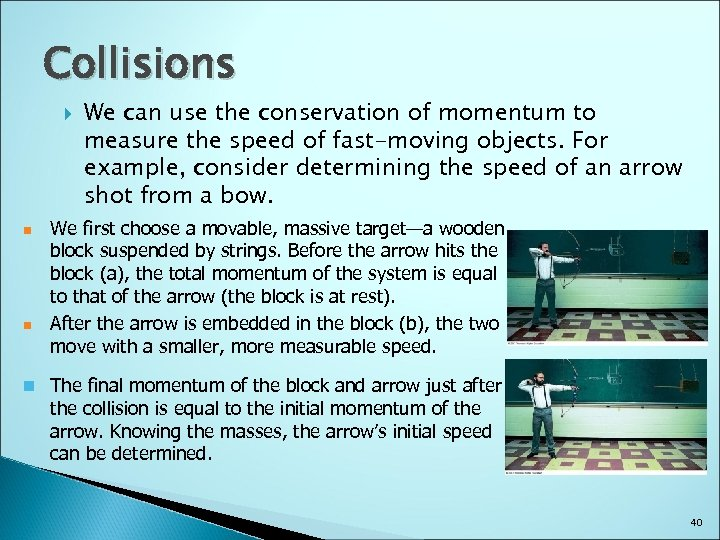Collisions n n We can use the conservation of momentum to measure the speed of fast-moving objects. For example, consider determining the speed of an arrow shot from a bow. We first choose a movable, massive target—a wooden block suspended by strings. Before the arrow hits the block (a), the total momentum of the system is equal to that of the arrow (the block is at rest). After the arrow is embedded in the block (b), the two move with a smaller, more measurable speed. n The final momentum of the block and arrow just after the collision is equal to the initial momentum of the arrow. Knowing the masses, the arrow’s initial speed can be determined. 40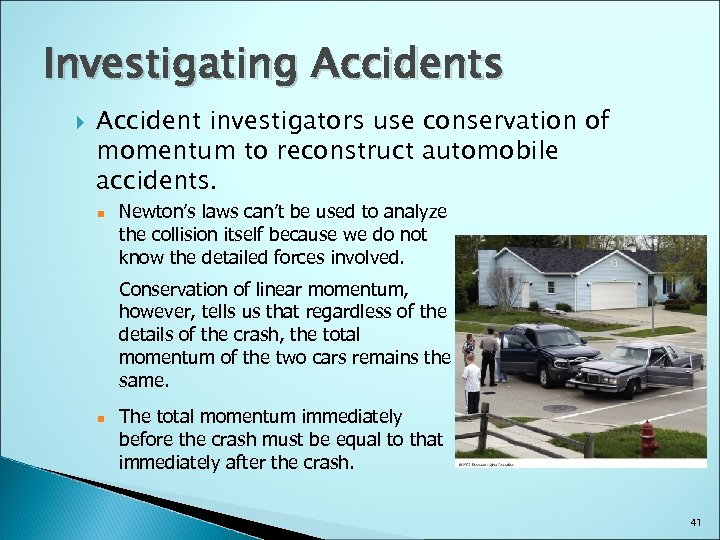Investigating Accidents Accident investigators use conservation of momentum to reconstruct automobile accidents. n Newton’s laws can’t be used to analyze the collision itself because we do not know the detailed forces involved. Conservation of linear momentum, however, tells us that regardless of the details of the crash, the total momentum of the two cars remains the same. n The total momentum immediately before the crash must be equal to that immediately after the crash. 41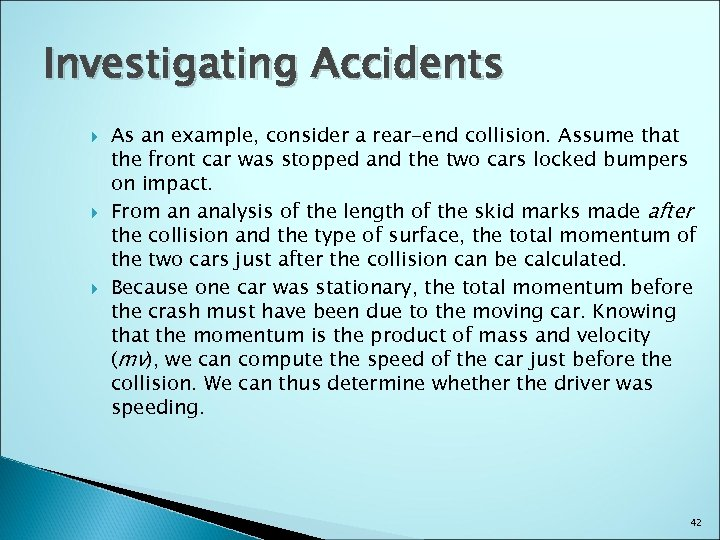Investigating Accidents As an example, consider a rear-end collision. Assume that the front car was stopped and the two cars locked bumpers on impact. From an analysis of the length of the skid marks made after the collision and the type of surface, the total momentum of the two cars just after the collision can be calculated. Because one car was stationary, the total momentum before the crash must have been due to the moving car. Knowing that the momentum is the product of mass and velocity (mv), we can compute the speed of the car just before the collision. We can thus determine whether the driver was speeding. 42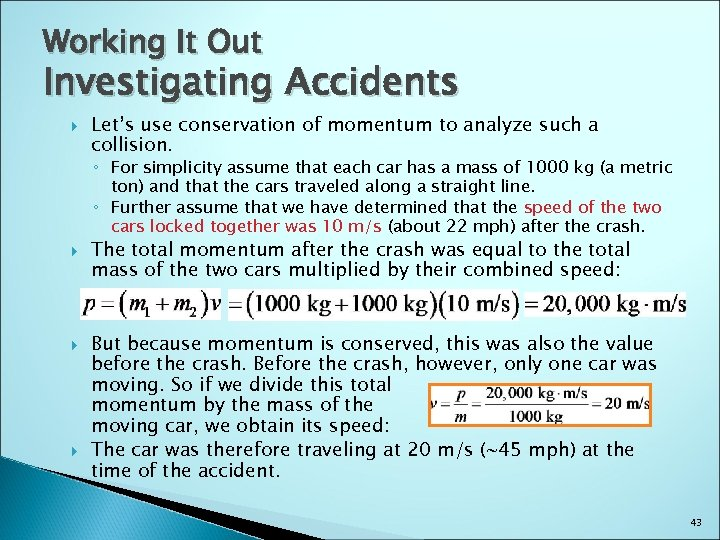Working It Out Investigating Accidents Let’s use conservation of momentum to analyze such a collision. ◦ For simplicity assume that each car has a mass of 1000 kg (a metric ton) and that the cars traveled along a straight line. ◦ Further assume that we have determined that the speed of the two cars locked together was 10 m/s (about 22 mph) after the crash. The total momentum after the crash was equal to the total mass of the two cars multiplied by their combined speed: But because momentum is conserved, this was also the value before the crash. Before the crash, however, only one car was moving. So if we divide this total momentum by the mass of the moving car, we obtain its speed: The car was therefore traveling at 20 m/s (~45 mph) at the time of the accident. 43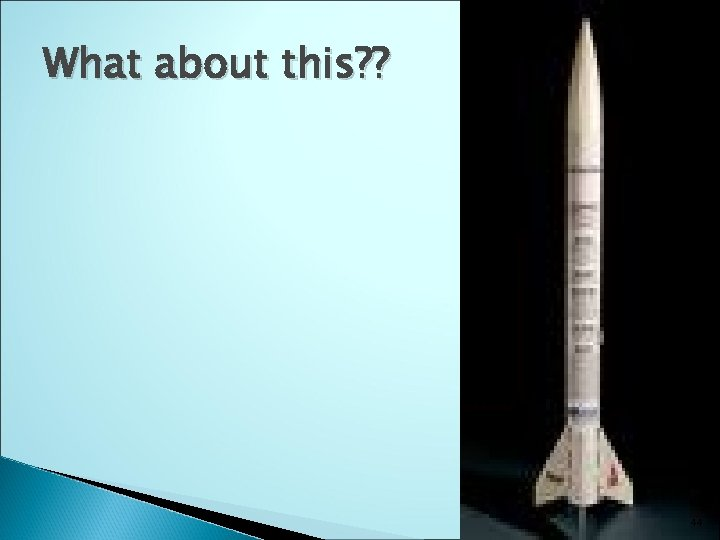What about this? ? 44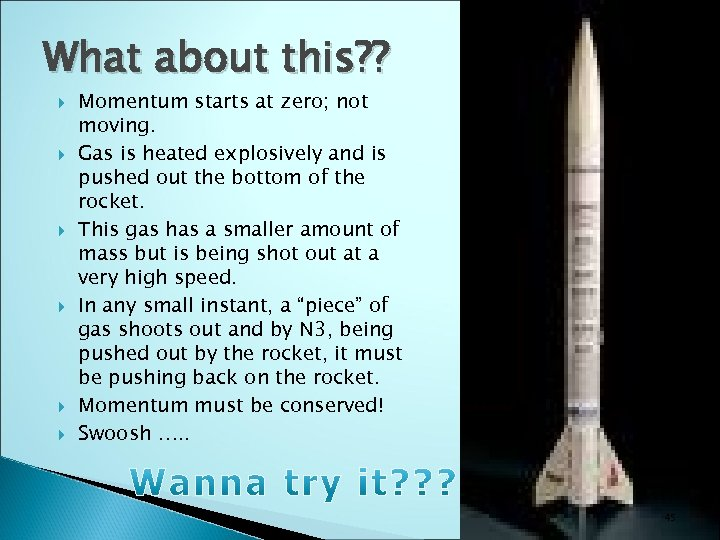What about this? ? Momentum starts at zero; not moving. Gas is heated explosively and is pushed out the bottom of the rocket. This gas has a smaller amount of mass but is being shot out at a very high speed. In any small instant, a “piece” of gas shoots out and by N 3, being pushed out by the rocket, it must be pushing back on the rocket. Momentum must be conserved! Swoosh …. . 45So now you know all about momentum! Why do we care? 46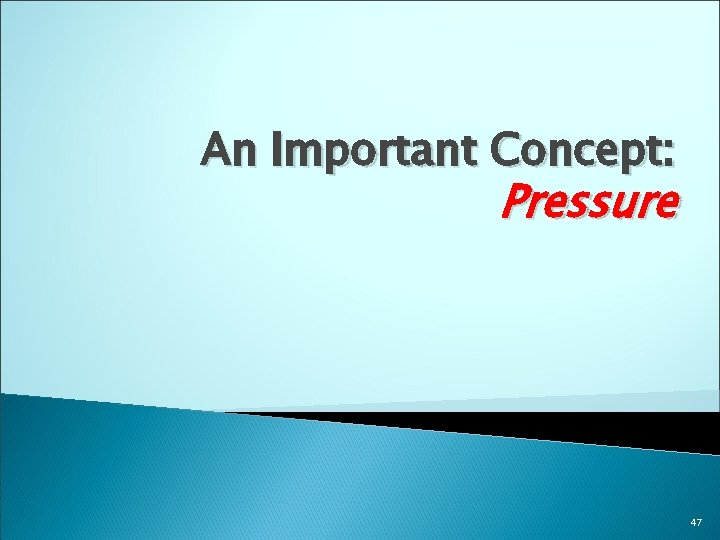An Important Concept: Pressure 47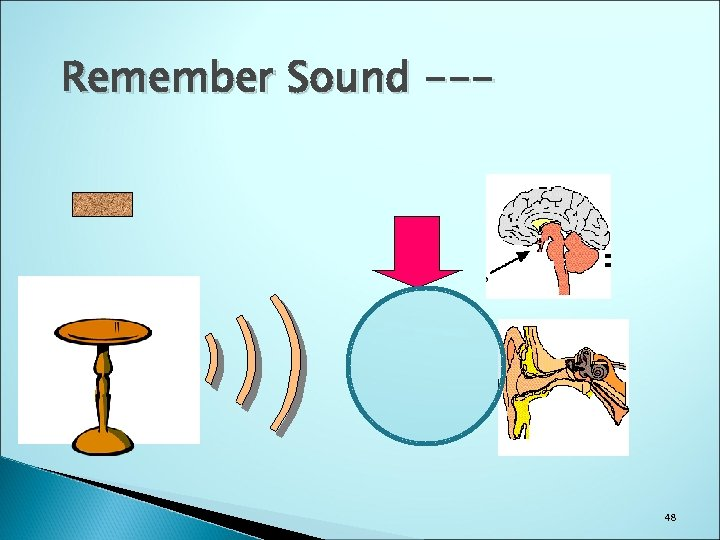Remember Sound --- 48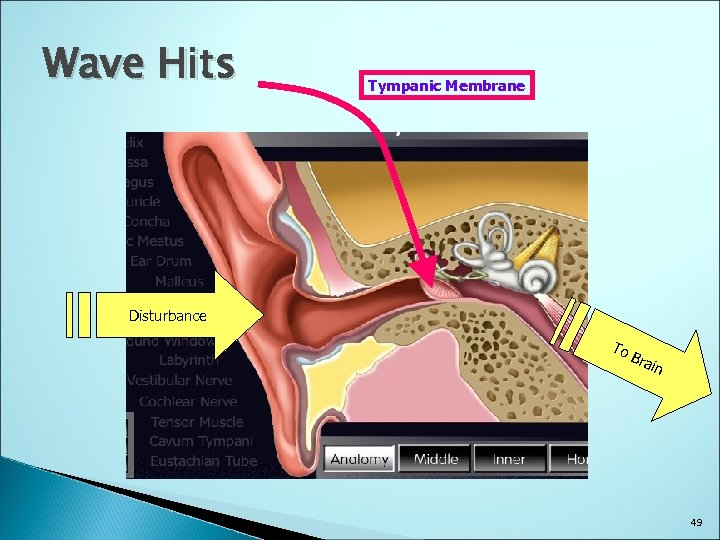Wave Hits Tympanic Membrane Disturbance To Bra in 49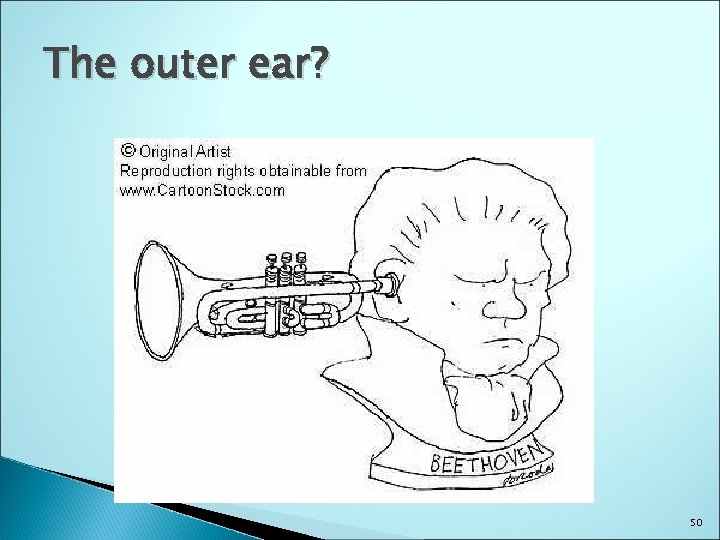The outer ear? 50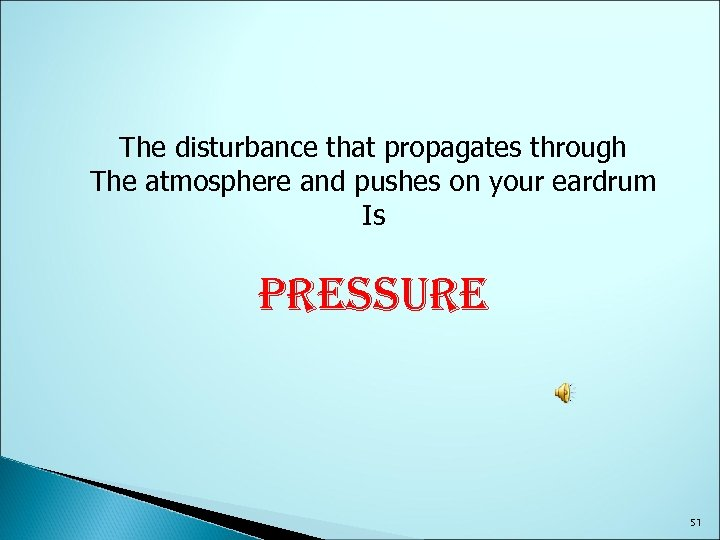The disturbance that propagates through The atmosphere and pushes on your eardrum Is PRESSURE 51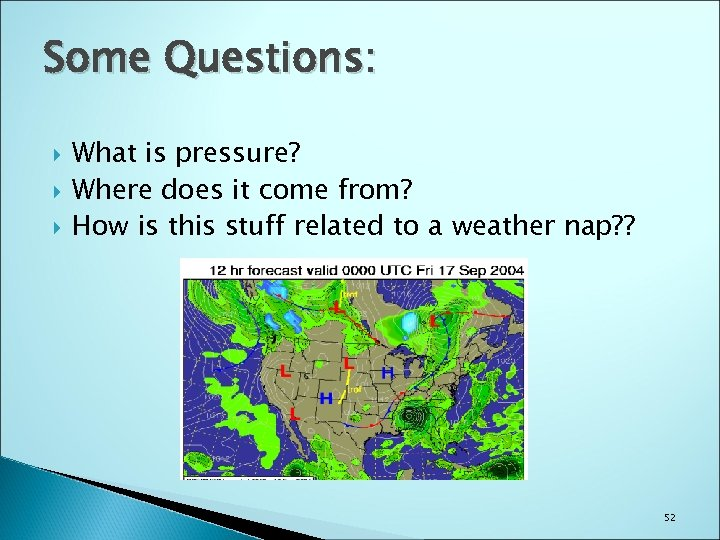Some Questions: What is pressure? Where does it come from? How is this stuff related to a weather nap? ? 52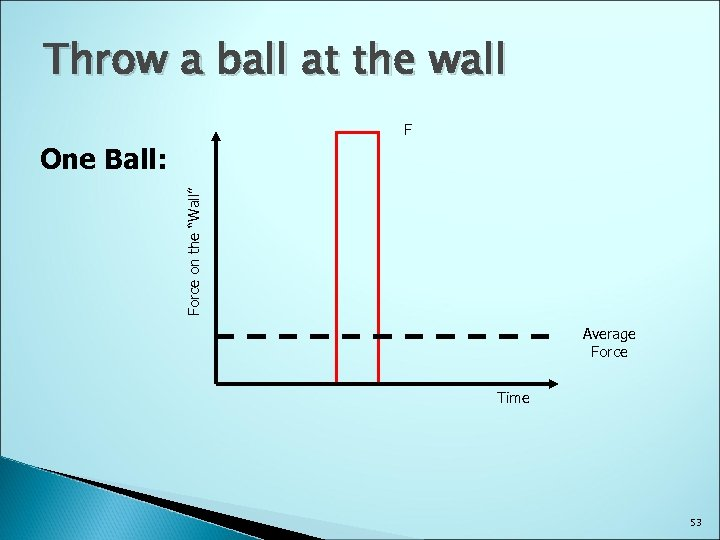Throw a ball at the wall F Force on the “Wall” One Ball: Average Force Time 53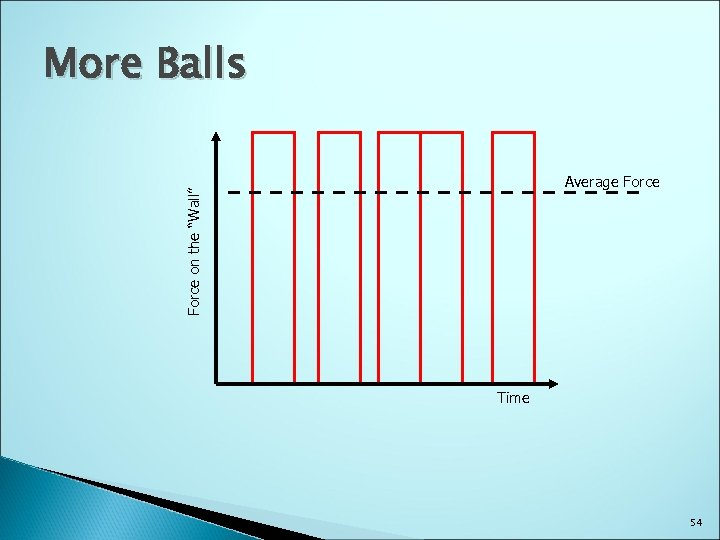More Balls Force on the “Wall” Average Force Time 54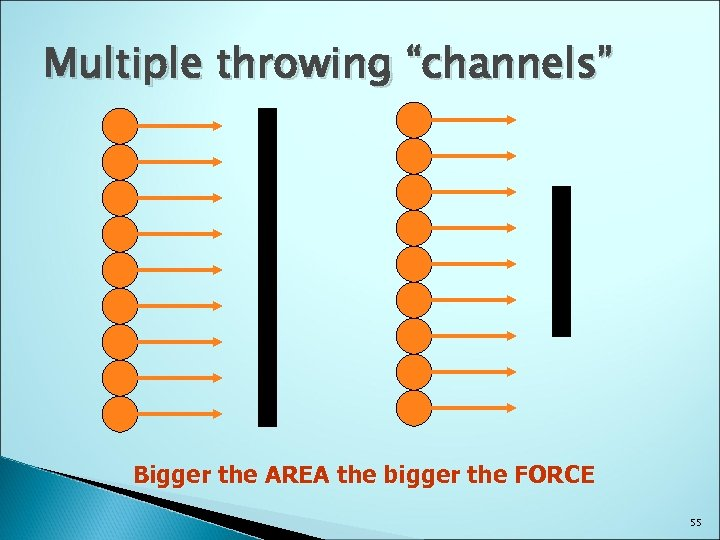Multiple throwing “channels” Bigger the AREA the bigger the FORCE 55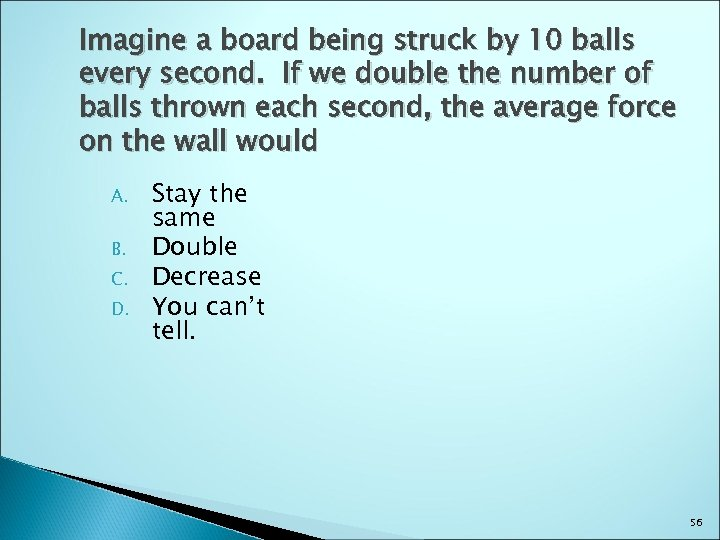Imagine a board being struck by 10 balls every second. If we double the number of balls thrown each second, the average force on the wall would A. B. C. D. Stay the same Double Decrease You can’t tell. 56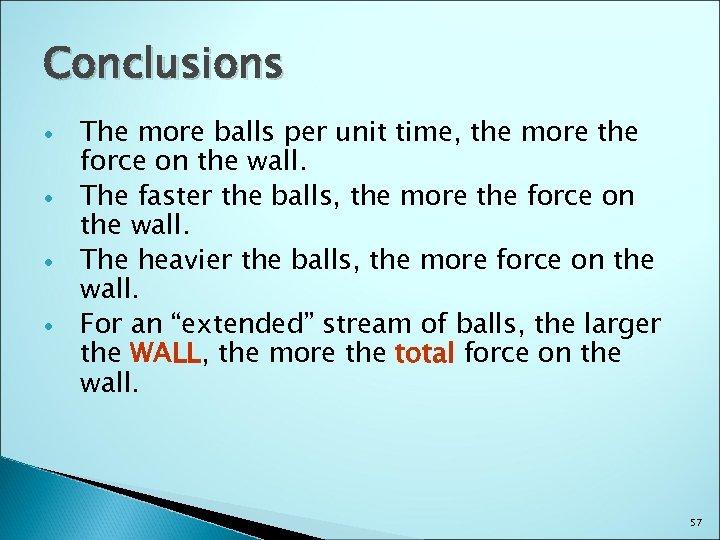Conclusions The more balls per unit time, the more the force on the wall. The faster the balls, the more the force on the wall. The heavier the balls, the more force on the wall. For an “extended” stream of balls, the larger the WALL, the more the total force on the WALL wall. 57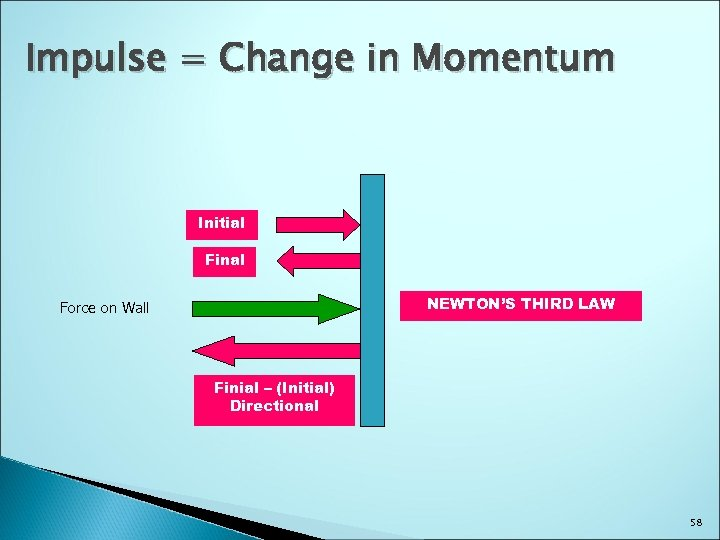Impulse = Change in Momentum Initial Final NEWTON’S THIRD LAW Force on Wall Finial – (Initial) Directional 58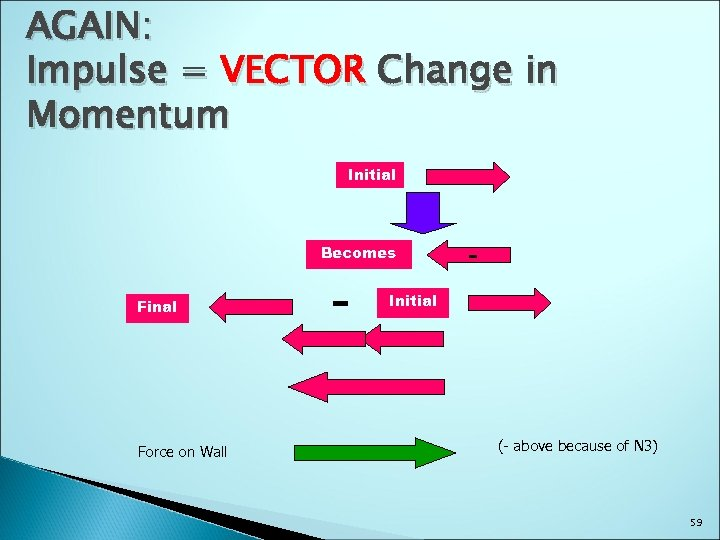AGAIN: Impulse = VECTOR Change in Momentum Initial Becomes Final Force on Wall - - Initial (- above because of N 3) 59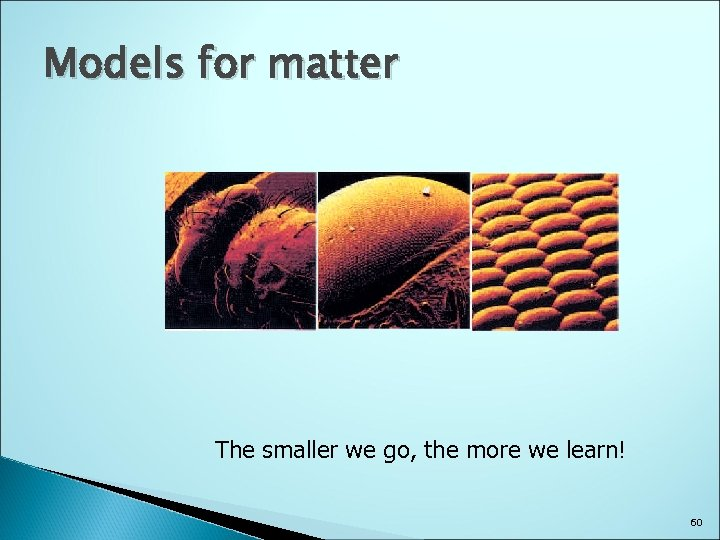Models for matter The smaller we go, the more we learn! 60Models often become reality We look to the outer limits of the universe. . We look at microbes We look at Virus particles We look at atoms We look at POLITICIANS VOTE often! Mc. Cain We Model it ALL! Obama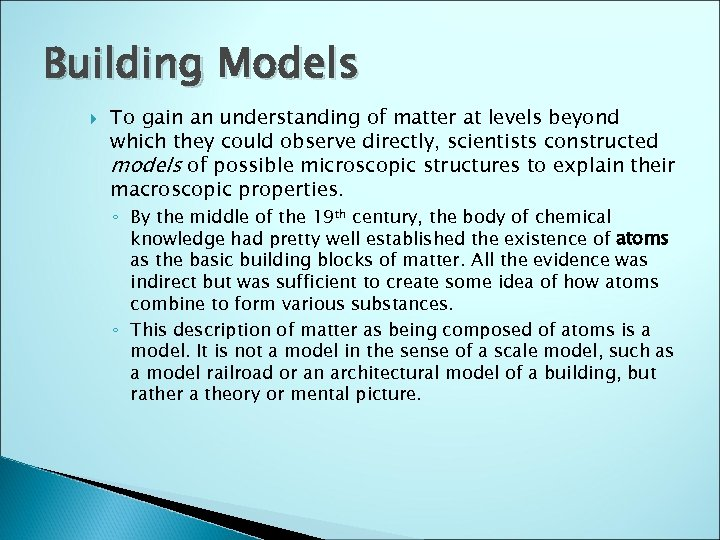Building Models To gain an understanding of matter at levels beyond which they could observe directly, scientists constructed models of possible microscopic structures to explain their macroscopic properties. ◦ By the middle of the 19 th century, the body of chemical knowledge had pretty well established the existence of atoms as the basic building blocks of matter. All the evidence was indirect but was sufficient to create some idea of how atoms combine to form various substances. ◦ This description of matter as being composed of atoms is a model. It is not a model in the sense of a scale model, such as a model railroad or an architectural model of a building, but rather a theory or mental picture.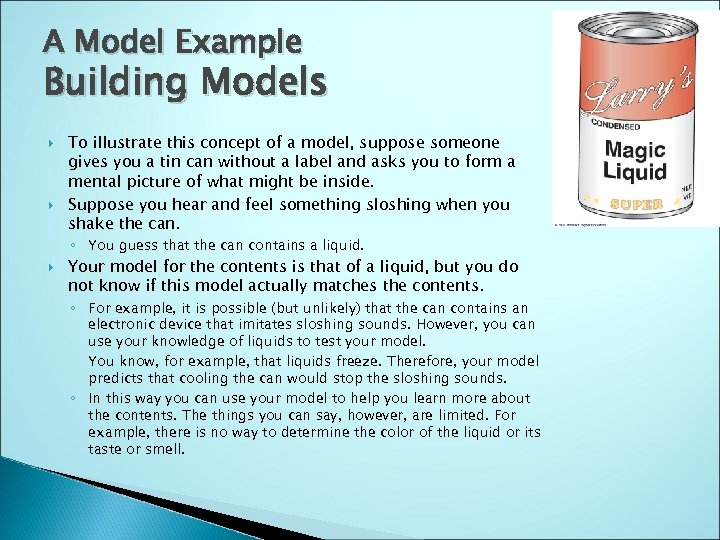A Model Example Building Models To illustrate this concept of a model, suppose someone gives you a tin can without a label and asks you to form a mental picture of what might be inside. Suppose you hear and feel something sloshing when you shake the can. ◦ You guess that the can contains a liquid. Your model for the contents is that of a liquid, but you do not know if this model actually matches the contents. ◦ For example, it is possible (but unlikely) that the can contains an electronic device that imitates sloshing sounds. However, you can use your knowledge of liquids to test your model. You know, for example, that liquids freeze. Therefore, your model predicts that cooling the can would stop the sloshing sounds. ◦ In this way you can use your model to help you learn more about the contents. The things you can say, however, are limited. For example, there is no way to determine the color of the liquid or its taste or smell.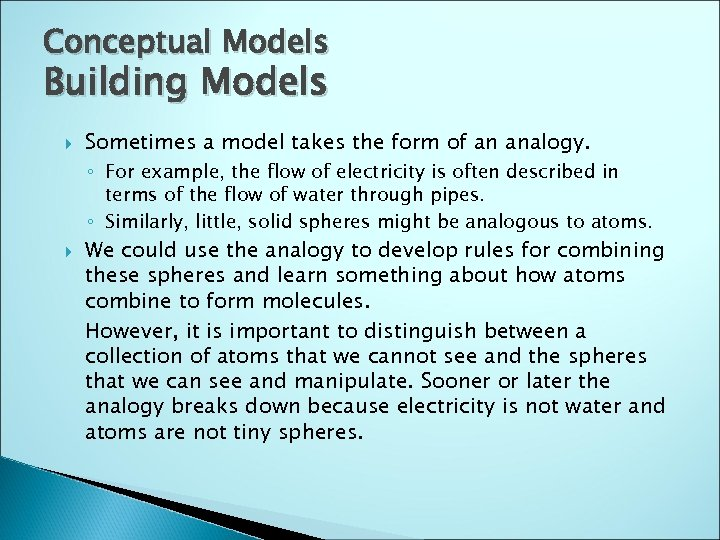Conceptual Models Building Models Sometimes a model takes the form of an analogy. ◦ For example, the flow of electricity is often described in terms of the flow of water through pipes. ◦ Similarly, little, solid spheres might be analogous to atoms. We could use the analogy to develop rules for combining these spheres and learn something about how atoms combine to form molecules. However, it is important to distinguish between a collection of atoms that we cannot see and the spheres that we can see and manipulate. Sooner or later the analogy breaks down because electricity is not water and atoms are not tiny spheres.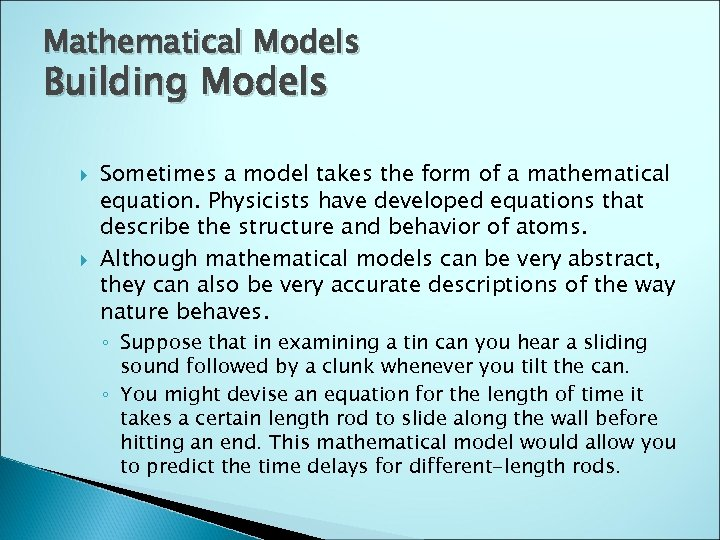Mathematical Models Building Models Sometimes a model takes the form of a mathematical equation. Physicists have developed equations that describe the structure and behavior of atoms. Although mathematical models can be very abstract, they can also be very accurate descriptions of the way nature behaves. ◦ Suppose that in examining a tin can you hear a sliding sound followed by a clunk whenever you tilt the can. ◦ You might devise an equation for the length of time it takes a certain length rod to slide along the wall before hitting an end. This mathematical model would allow you to predict the time delays for different-length rods.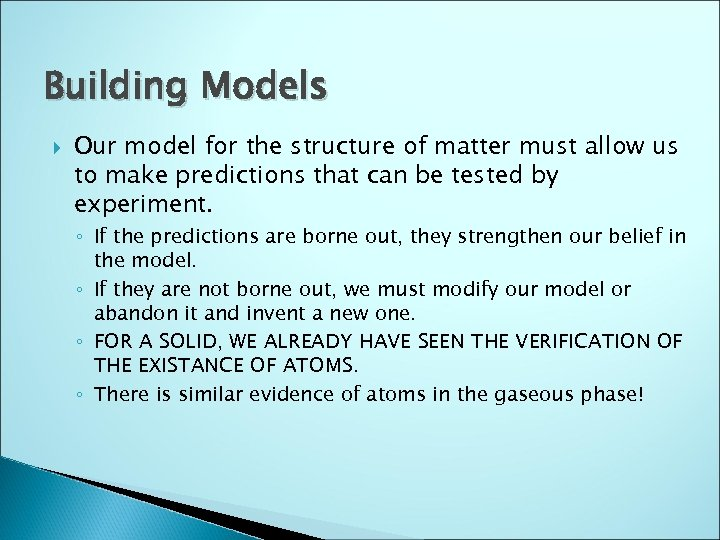Building Models Our model for the structure of matter must allow us to make predictions that can be tested by experiment. ◦ If the predictions are borne out, they strengthen our belief in the model. ◦ If they are not borne out, we must modify our model or abandon it and invent a new one. ◦ FOR A SOLID, WE ALREADY HAVE SEEN THE VERIFICATION OF THE EXISTANCE OF ATOMS. ◦ There is similar evidence of atoms in the gaseous phase!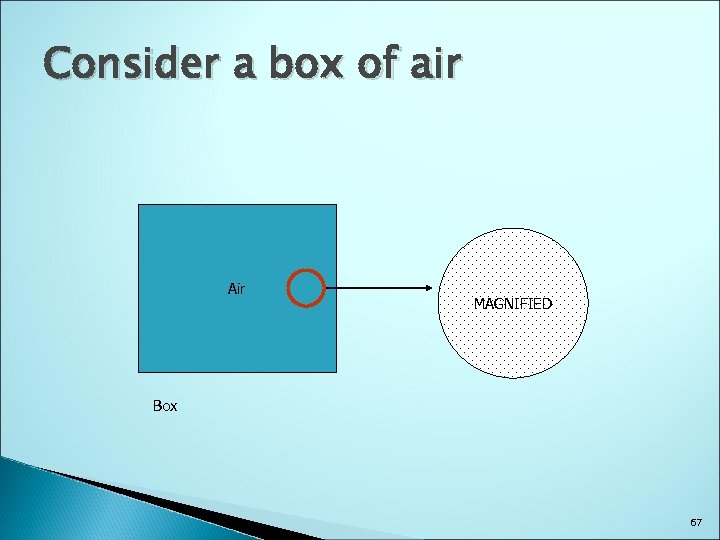Consider a box of air Air MAGNIFIED Box 67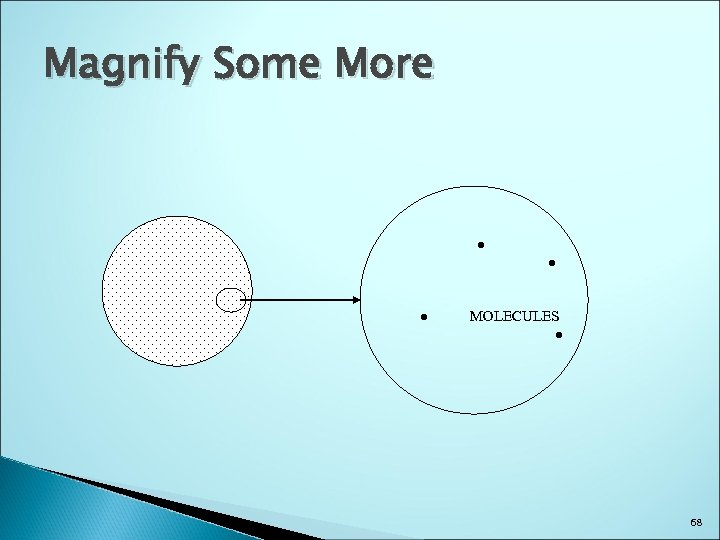Magnify Some More ● ● ● MOLECULES ● 68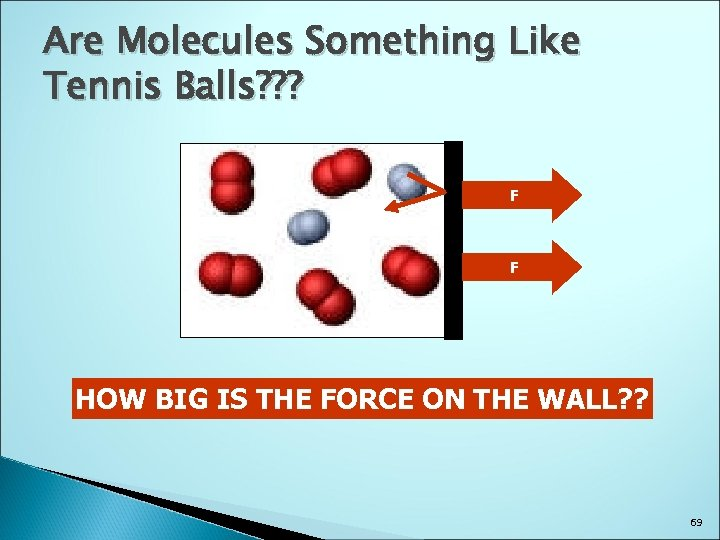Are Molecules Something Like Tennis Balls? ? ? F F HOW BIG IS THE FORCE ON THE WALL? ? 69http: //www. youtube. com/watch? v=ni. Wz. FSds 8 SY 70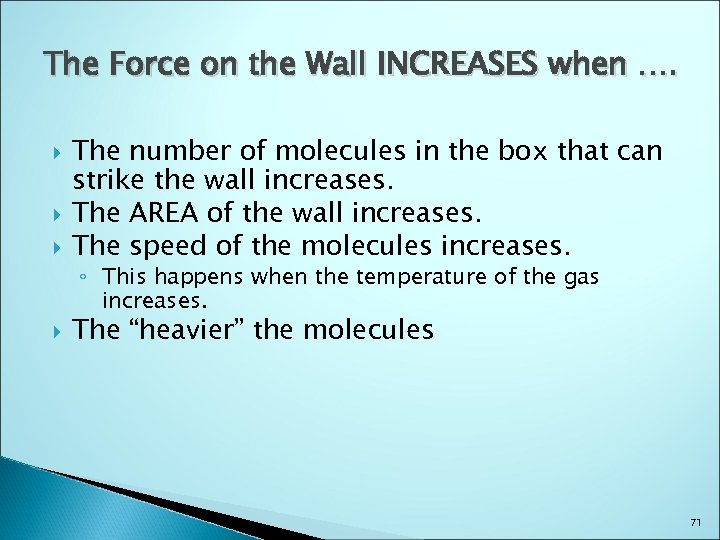The Force on the Wall INCREASES when …. The number of molecules in the box that can strike the wall increases. The AREA of the wall increases. The speed of the molecules increases. ◦ This happens when the temperature of the gas increases. The “heavier” the molecules 71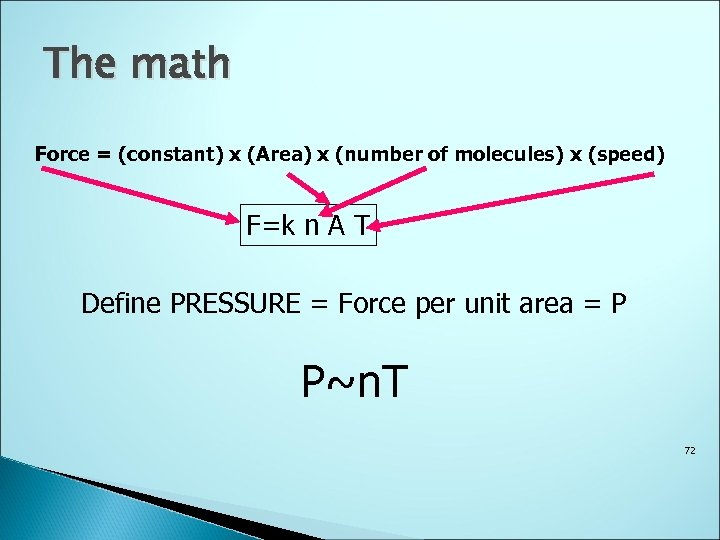The math Force = (constant) x (Area) x (number of molecules) x (speed) F=k n A T Define PRESSURE = Force per unit area = P P~n. T 72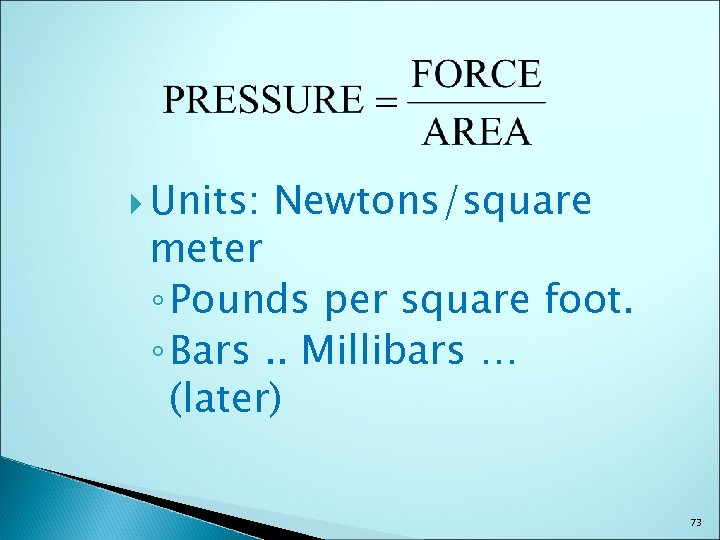Units: Newtons/square meter ◦ Pounds per square foot. ◦ Bars. . Millibars … (later) 73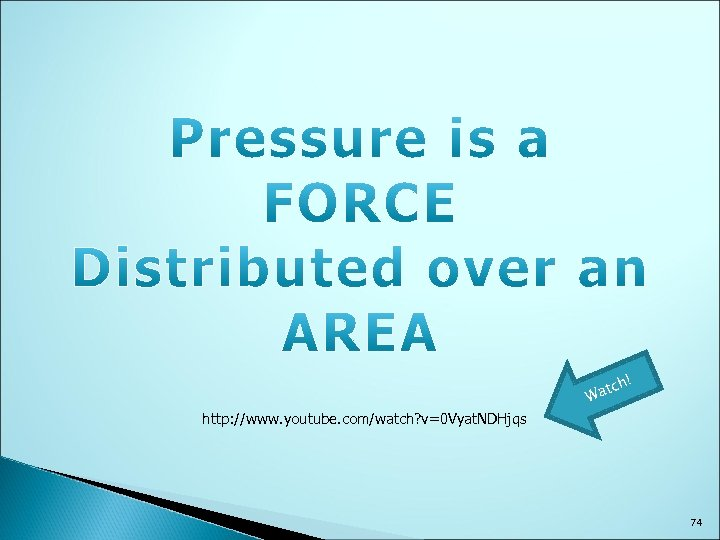http: //www. youtube. com/watch? v=0 Vyat. NDHjqs h! atc W 74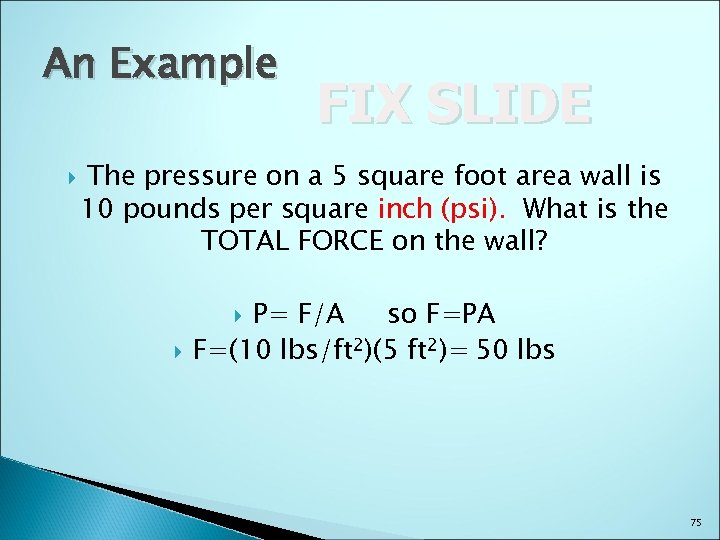An Example FIX SLIDE The pressure on a 5 square foot area wall is 10 pounds per square inch (psi). What is the TOTAL FORCE on the wall? P= F/A so F=PA F=(10 lbs/ft 2)(5 ft 2)= 50 lbs 75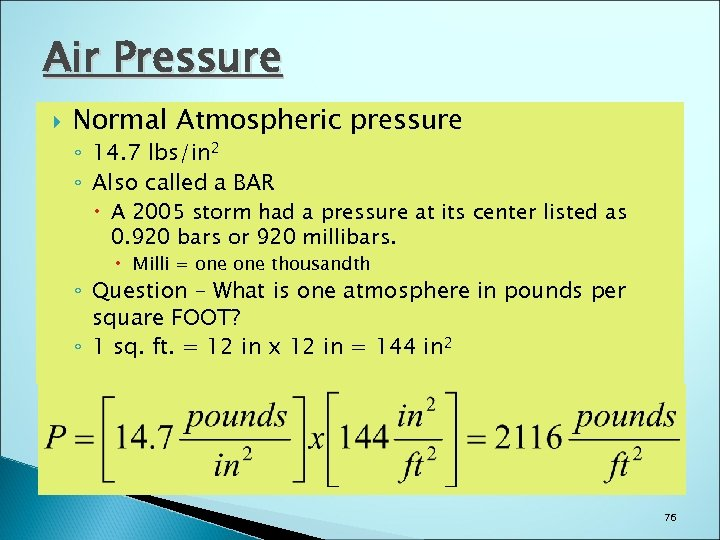Air Pressure Normal Atmospheric pressure ◦ 14. 7 lbs/in 2 ◦ Also called a BAR A 2005 storm had a pressure at its center listed as 0. 920 bars or 920 millibars. Milli = one thousandth ◦ Question – What is one atmosphere in pounds per square FOOT? ◦ 1 sq. ft. = 12 in x 12 in = 144 in 2 76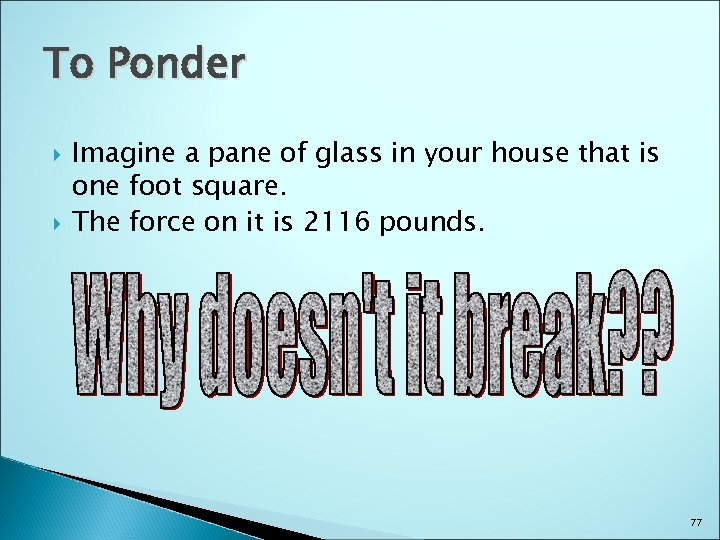To Ponder Imagine a pane of glass in your house that is one foot square. The force on it is 2116 pounds. 77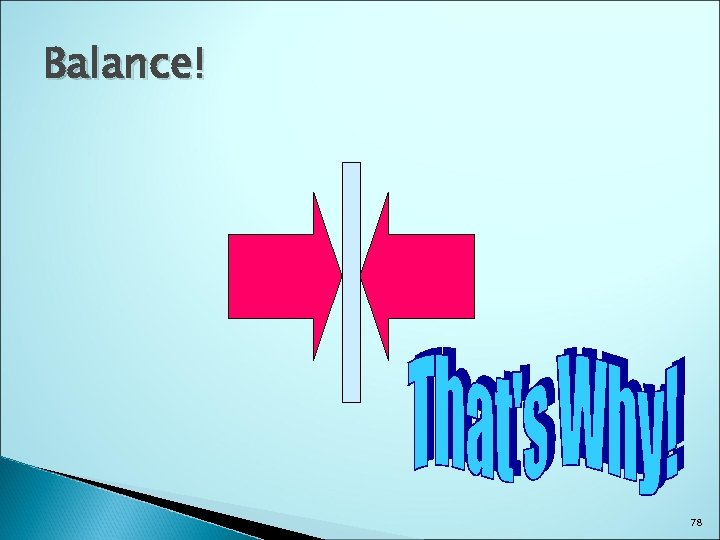Balance! 78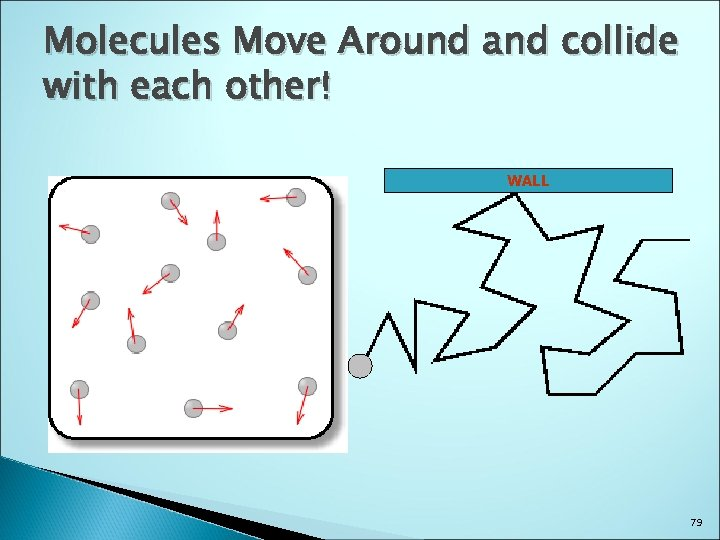Molecules Move Around and collide with each other! WALL 79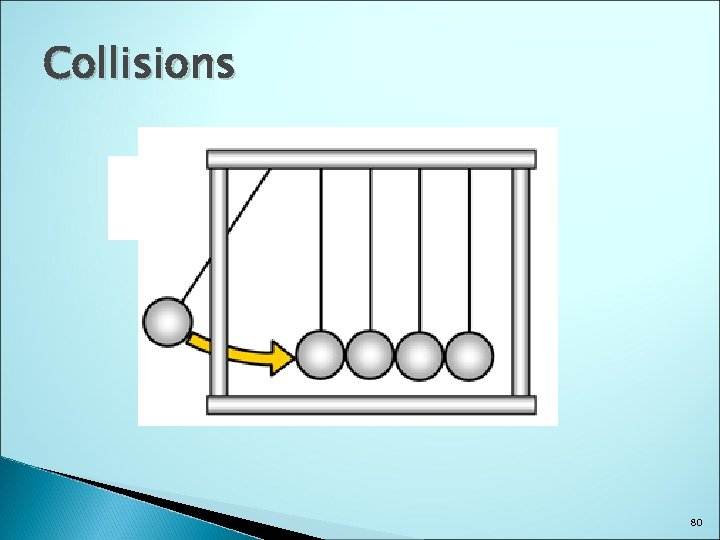Collisions 80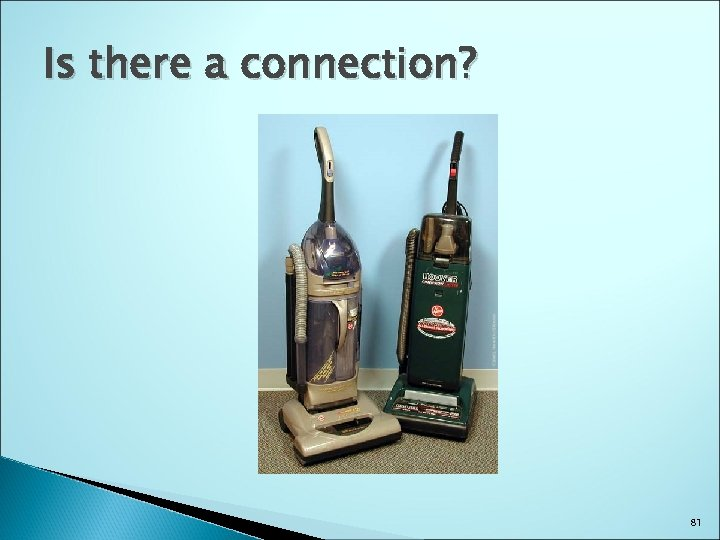Is there a connection? 81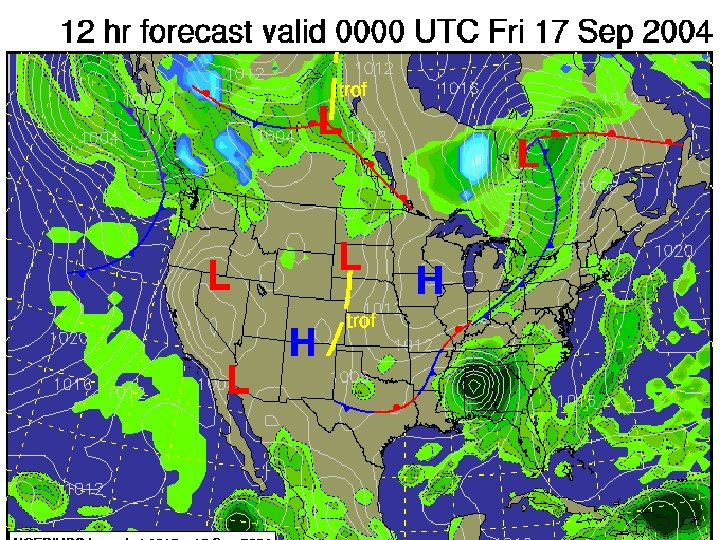82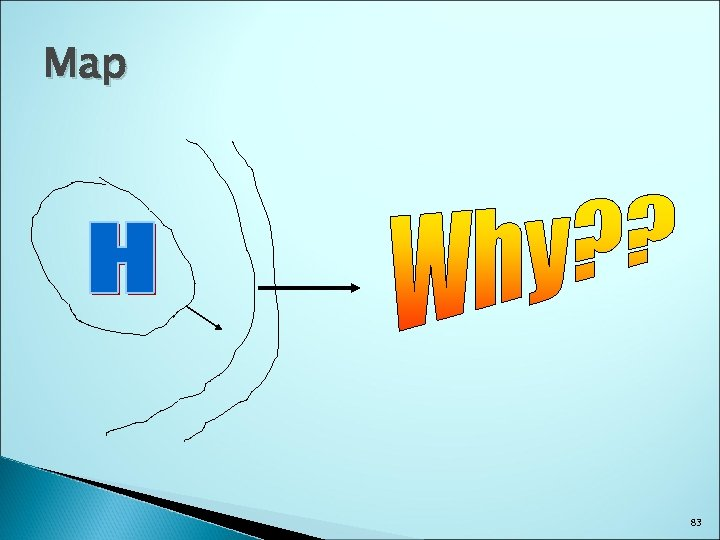Map 83Next – ENERGY a very important topic 84